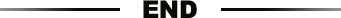# 成年人行走江湖，必须要懂的50句潜台词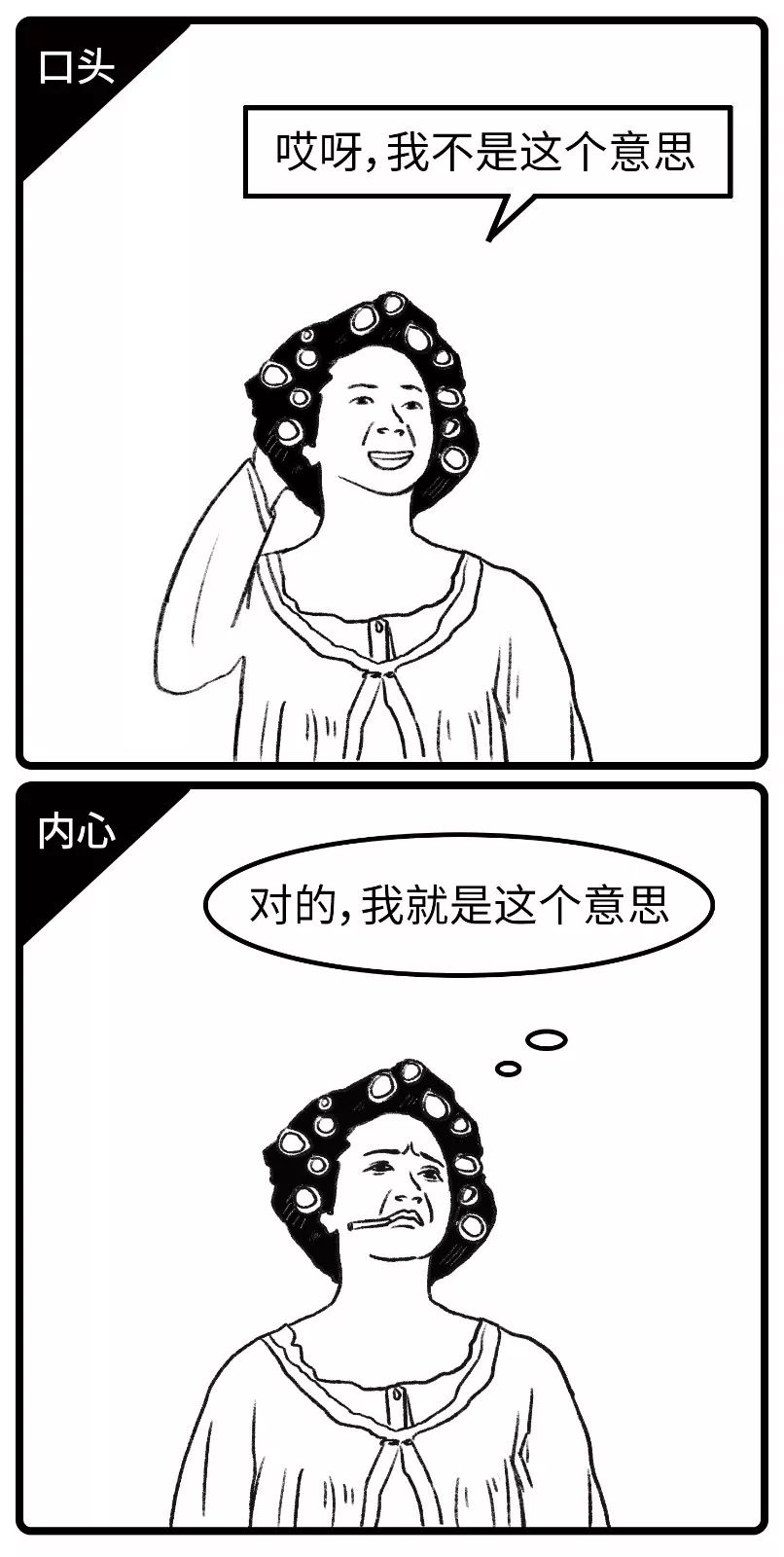01 呵呵

=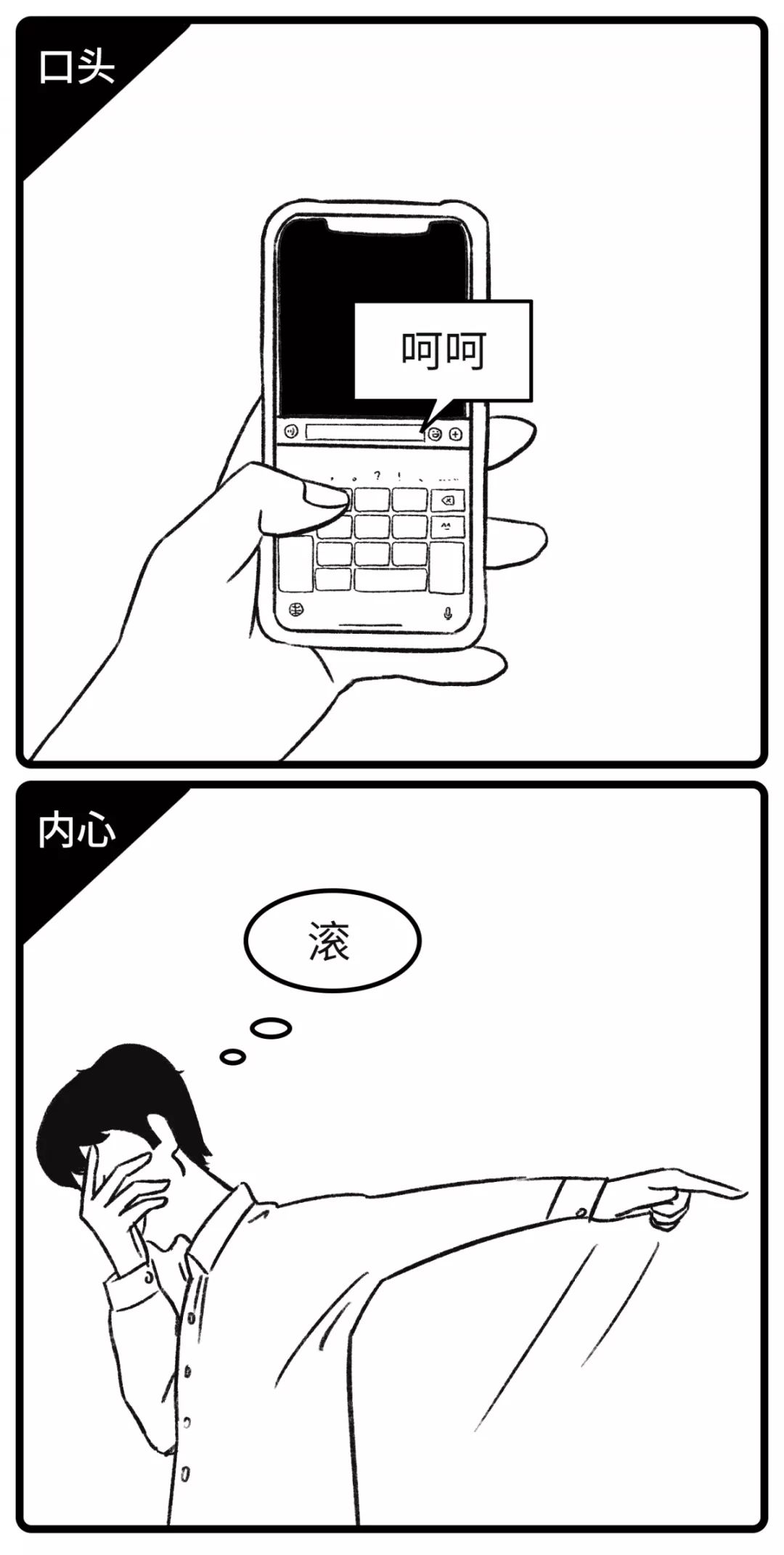02 好吧

=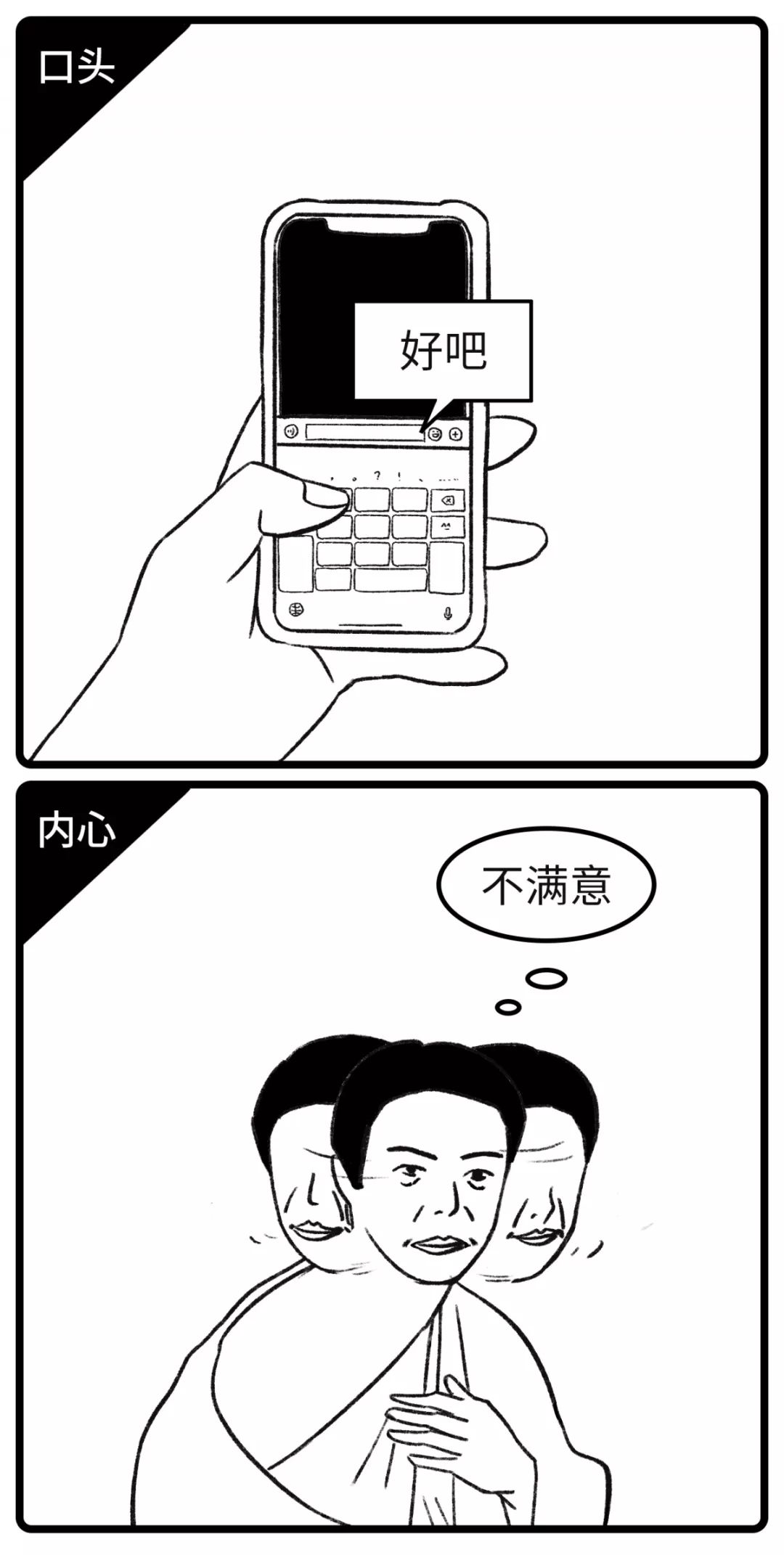03 我累了

=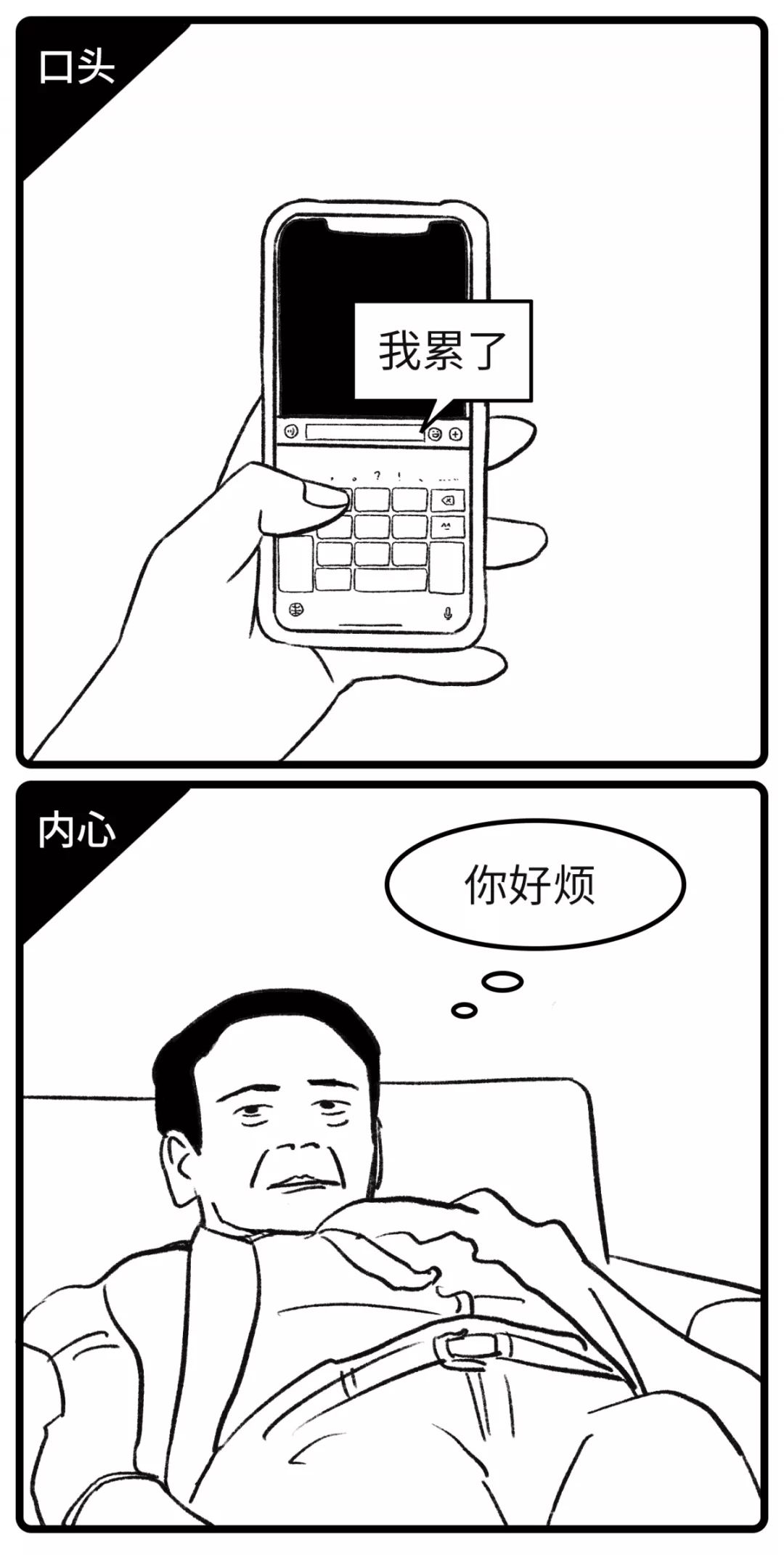04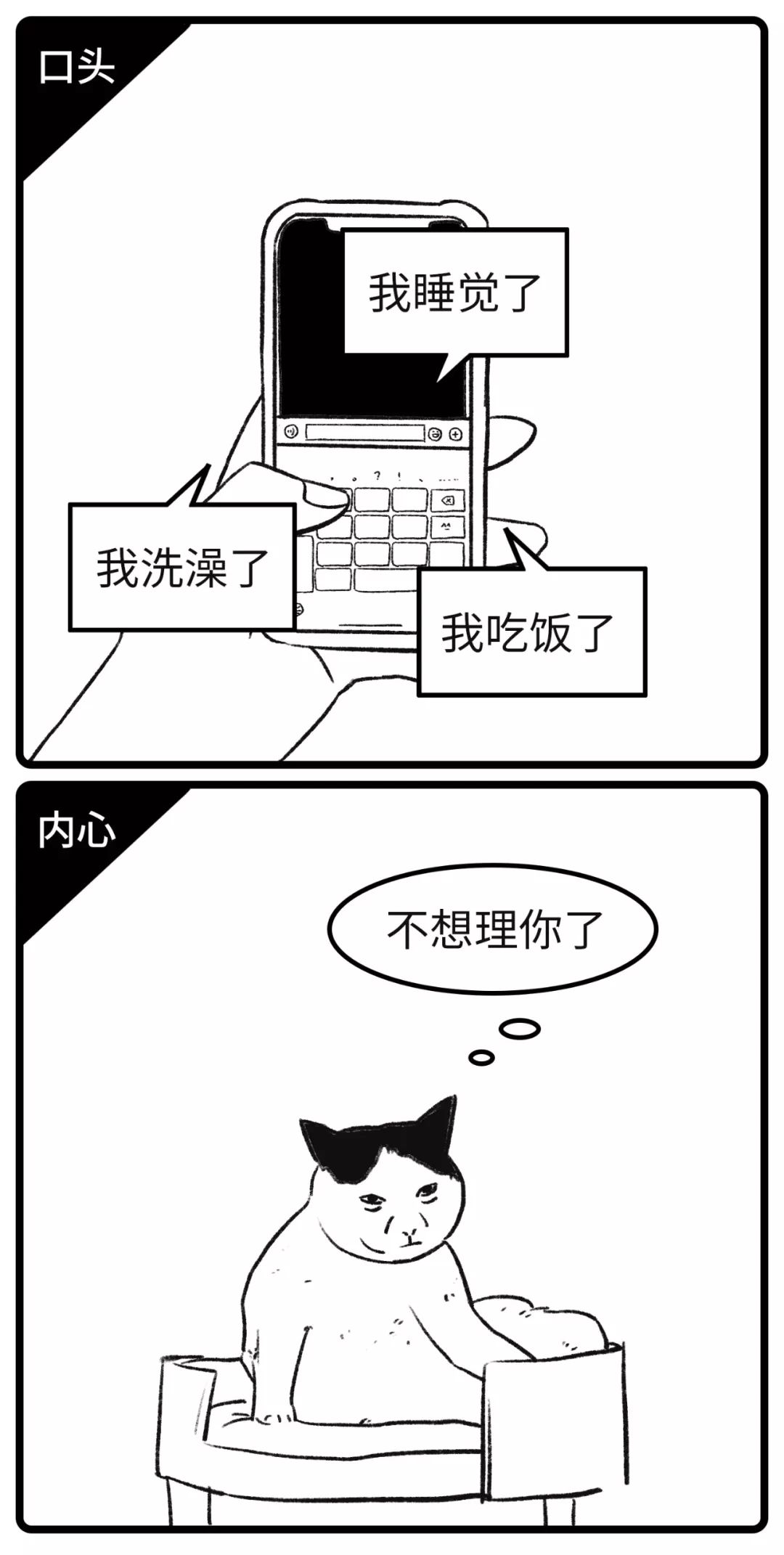05 你在干嘛

=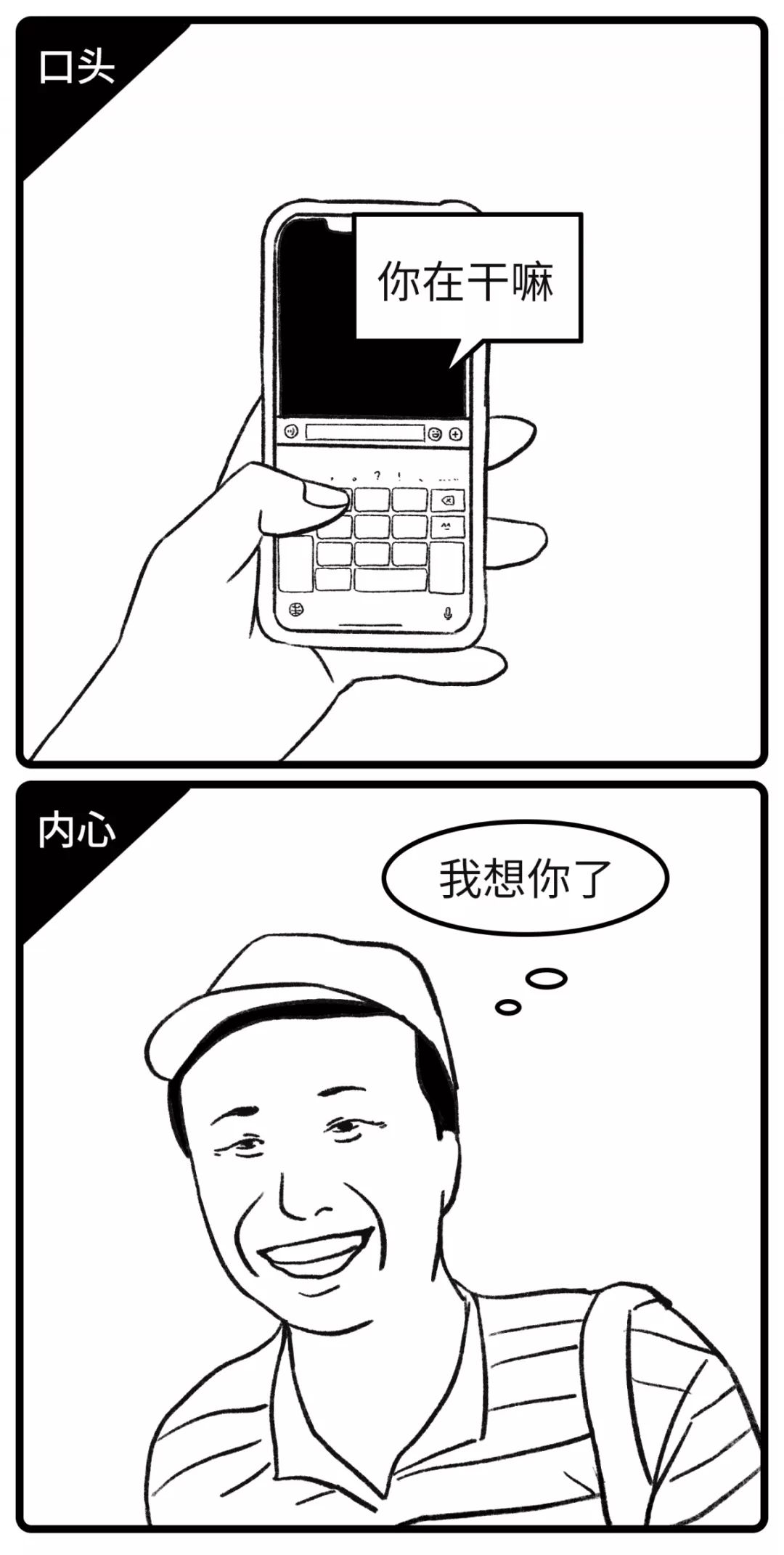06 有空再聊

=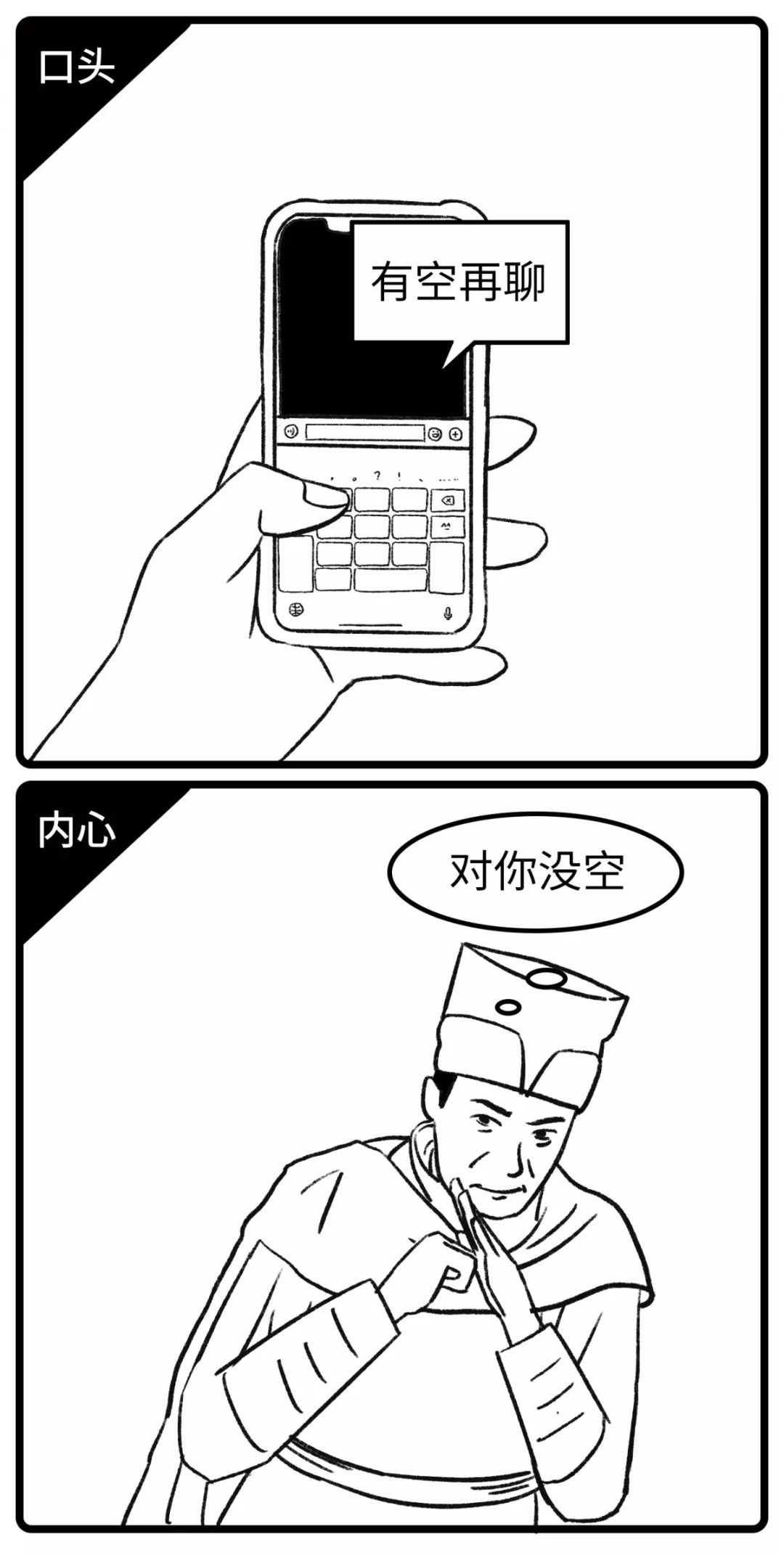07 等有时间聚聚

=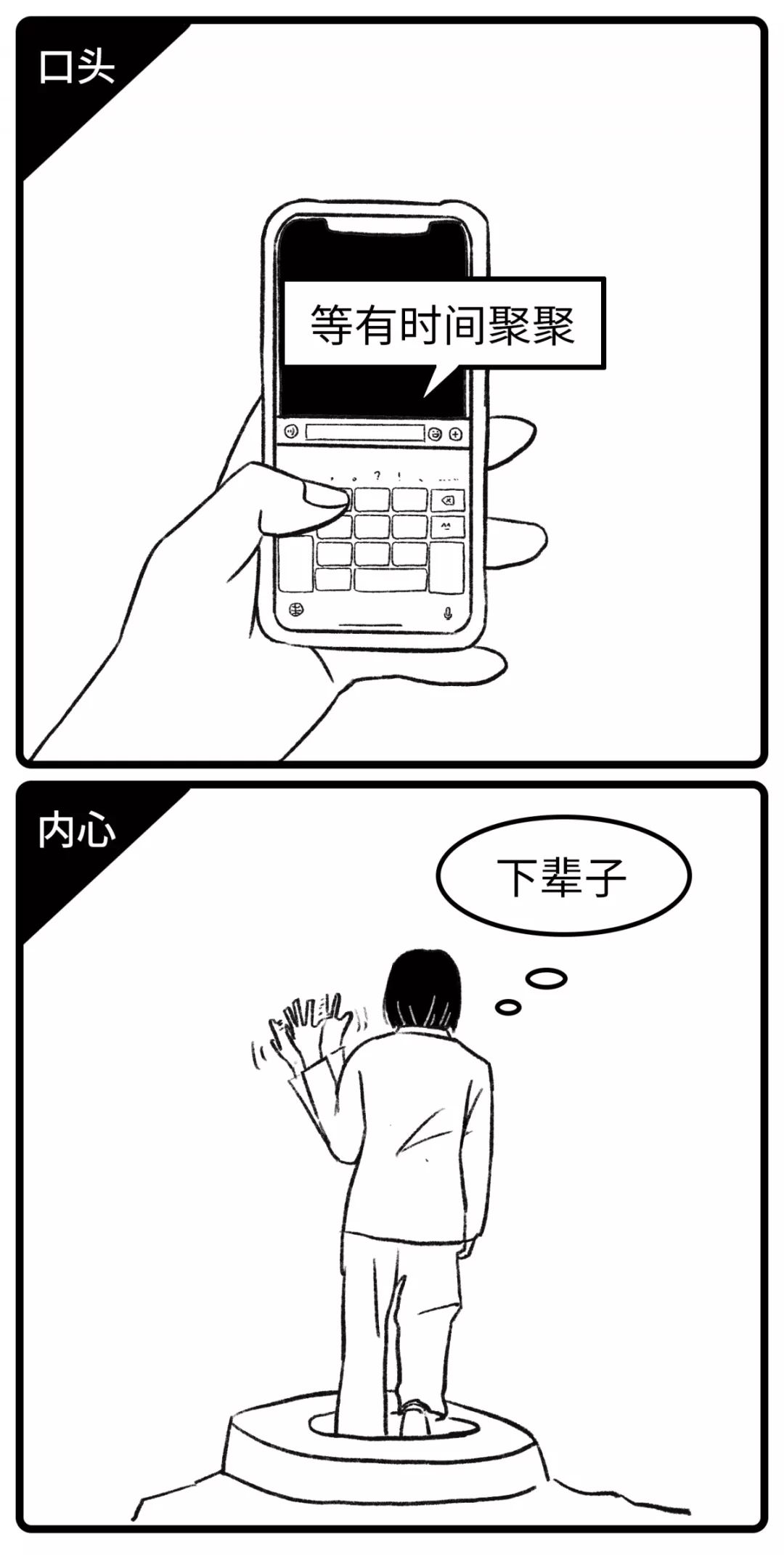08 哦

=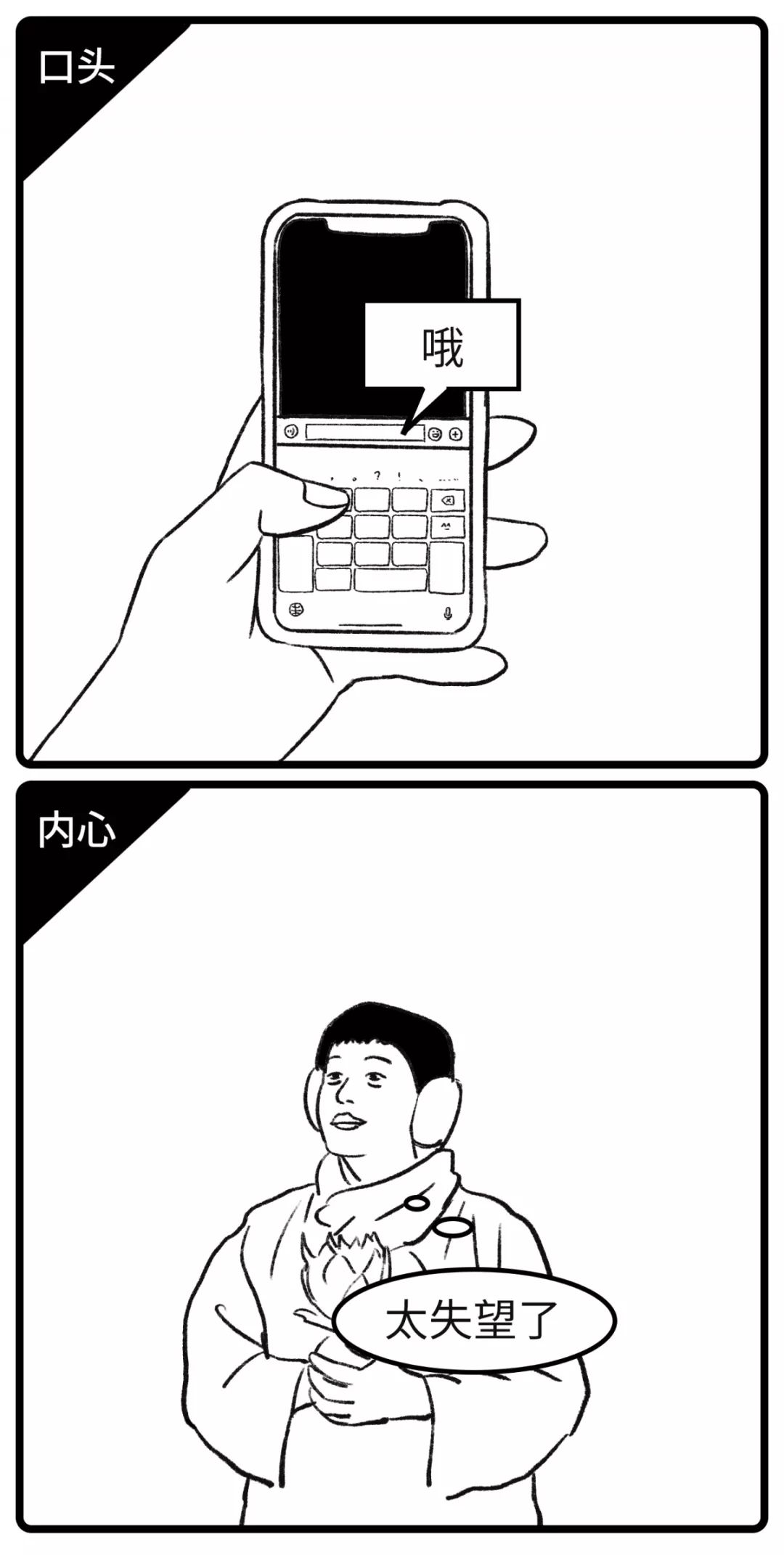09 ?

=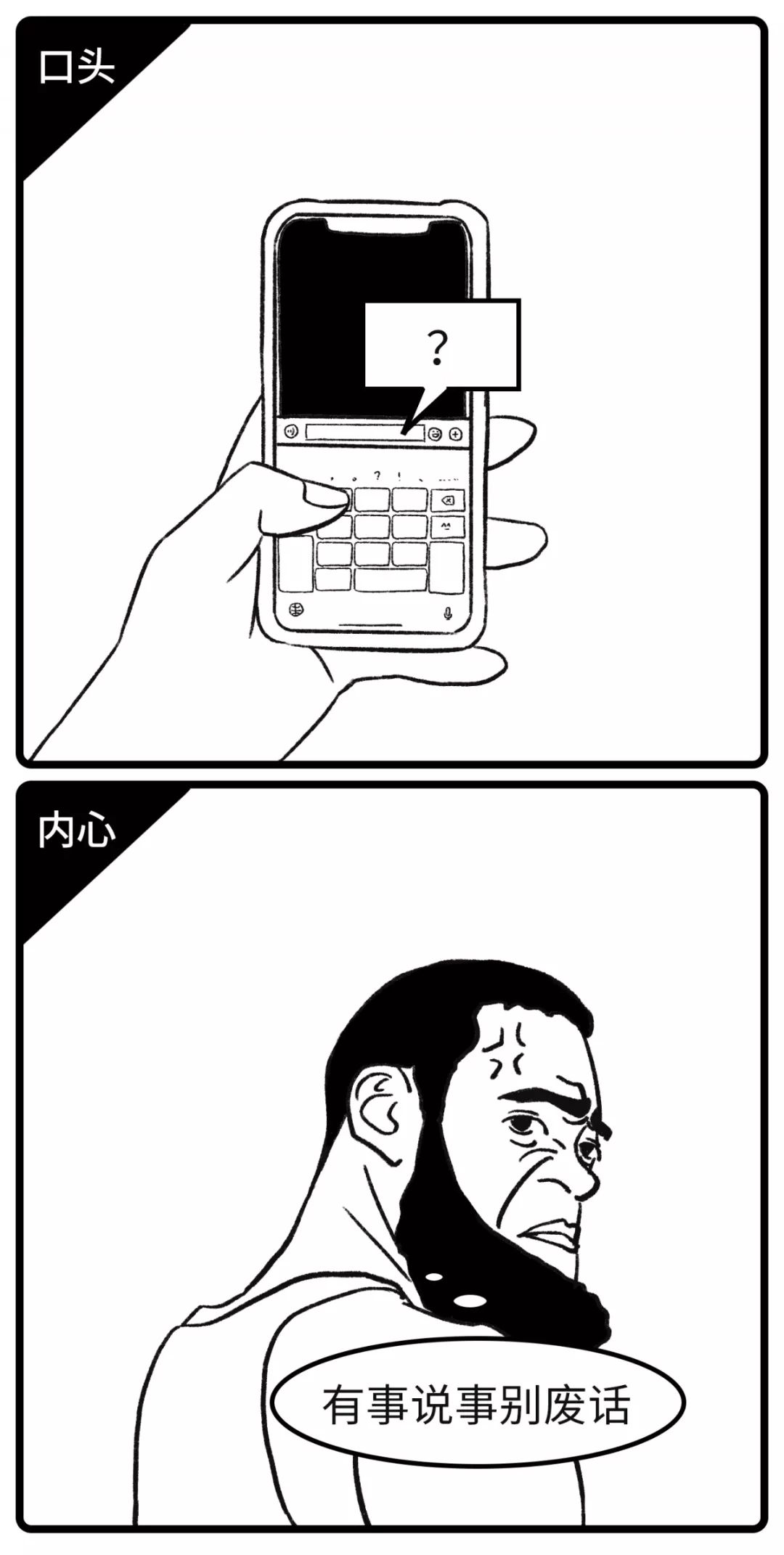10 以后再说吧

=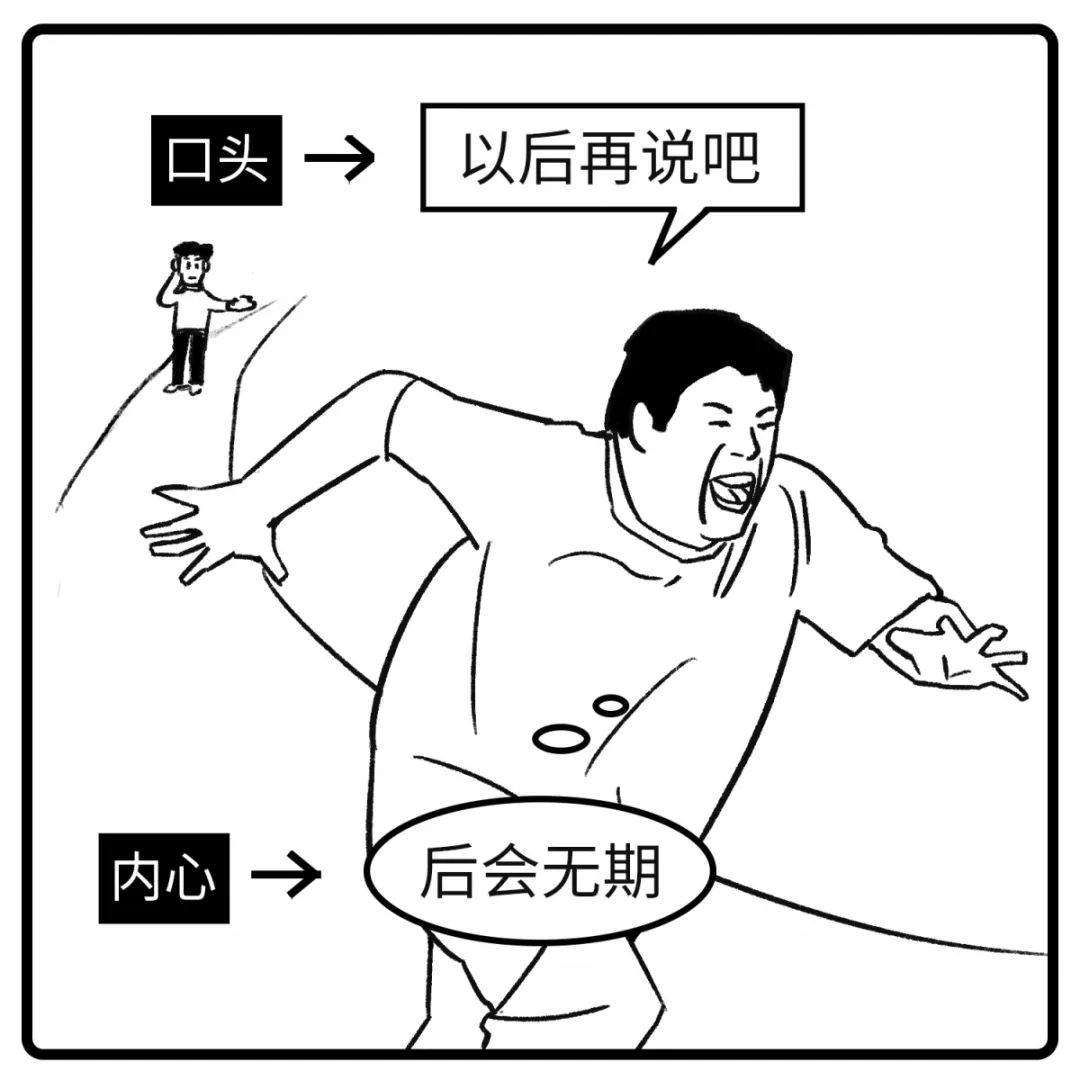11 你是个好人

=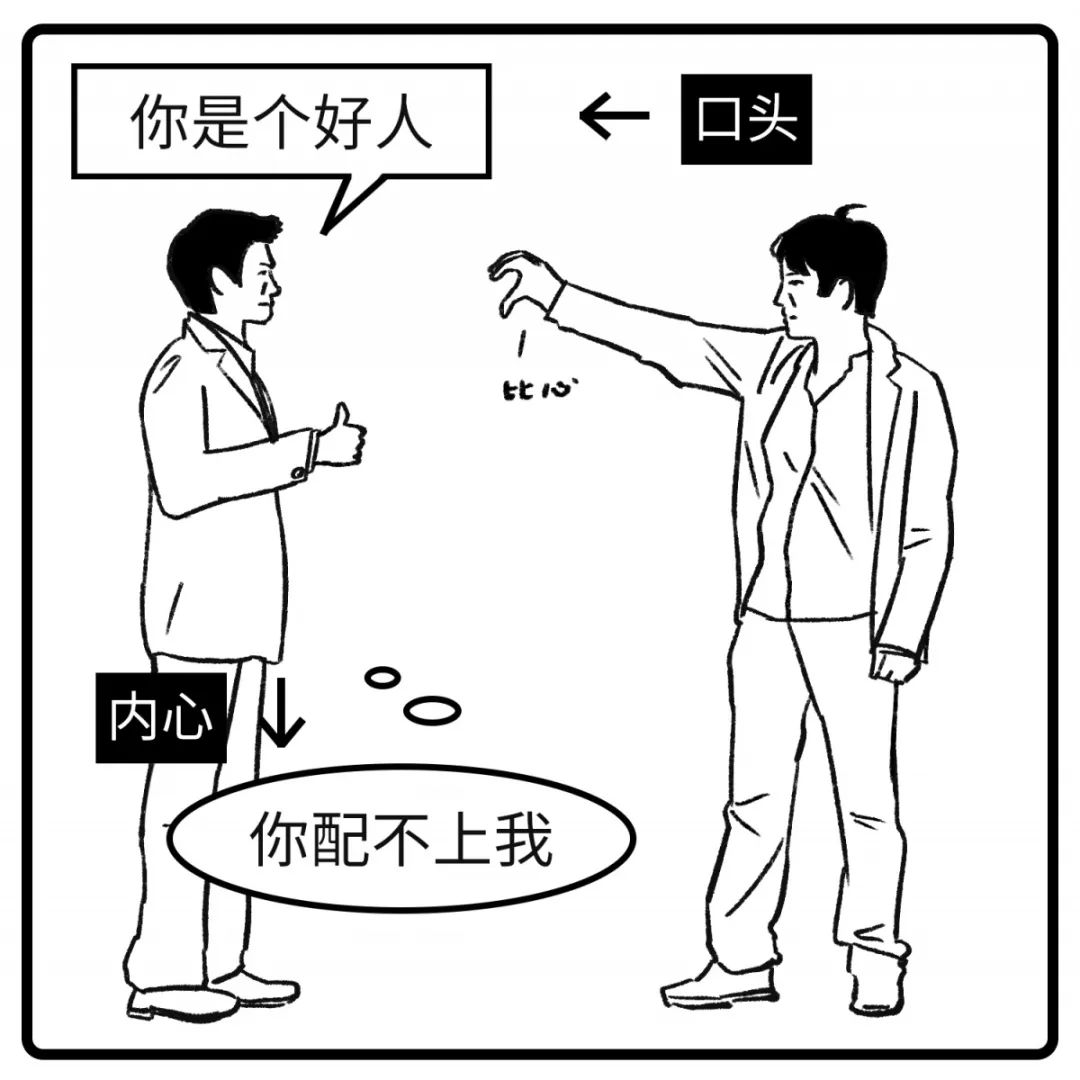12 我现在不想谈恋爱

=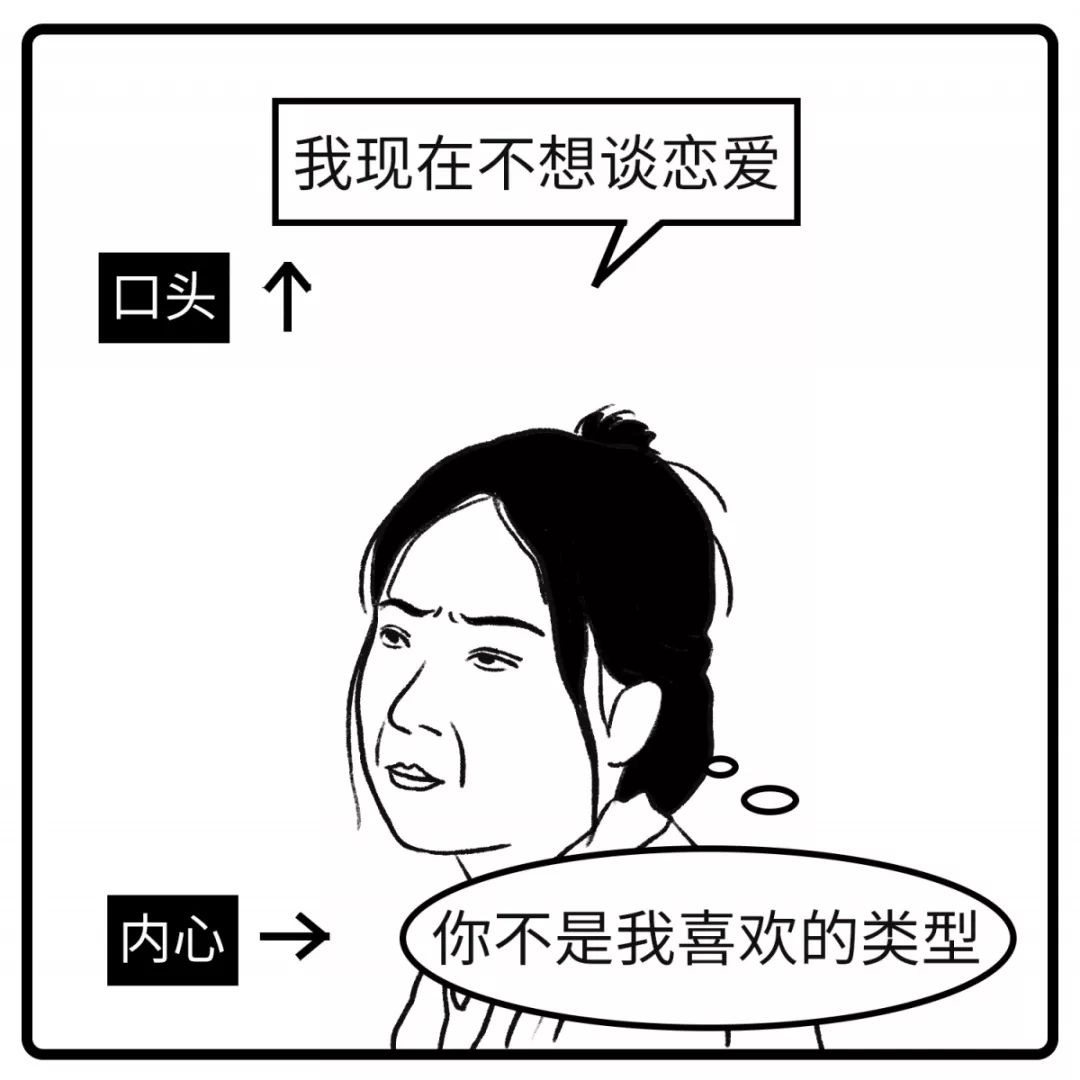-13-

=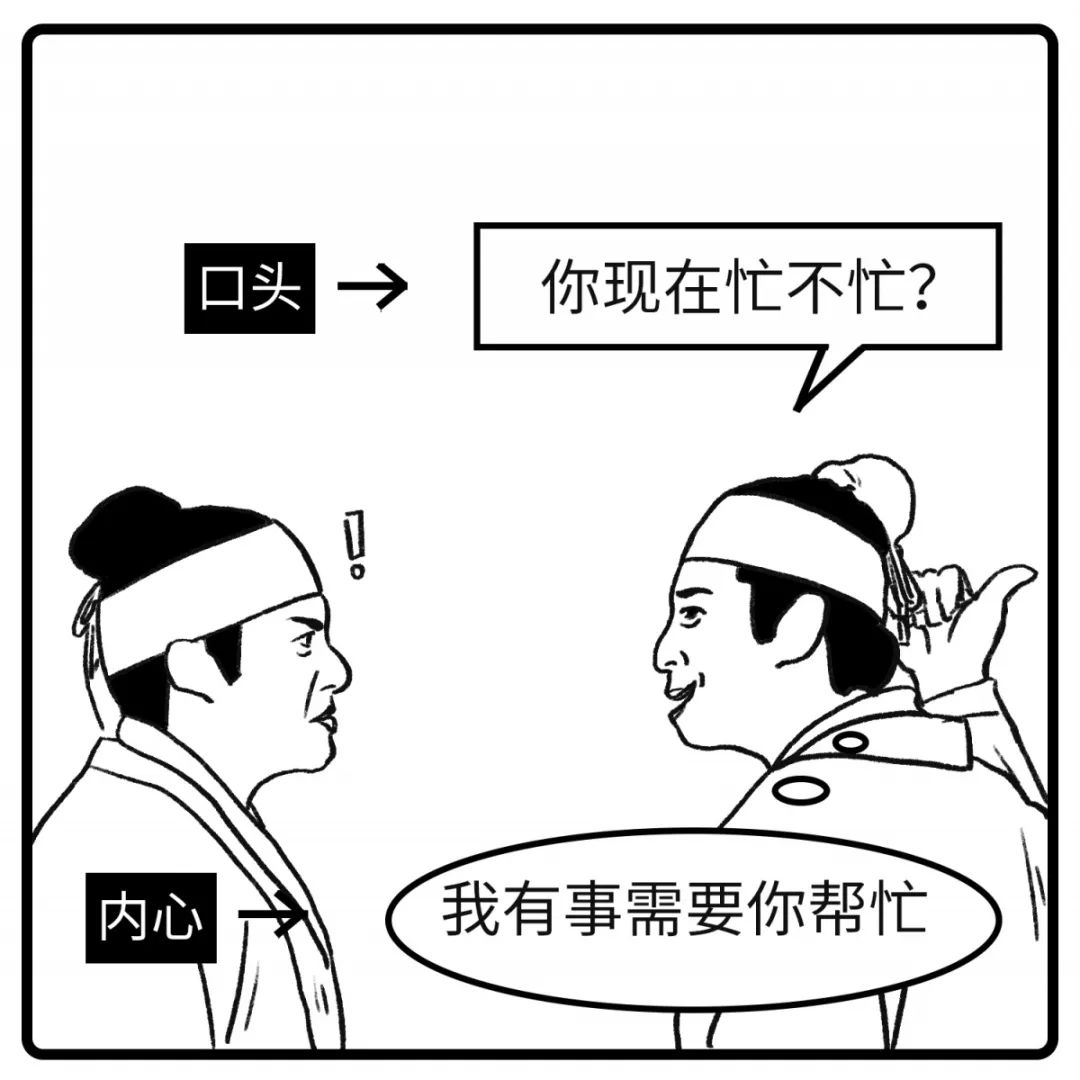14 最近怎么样？

=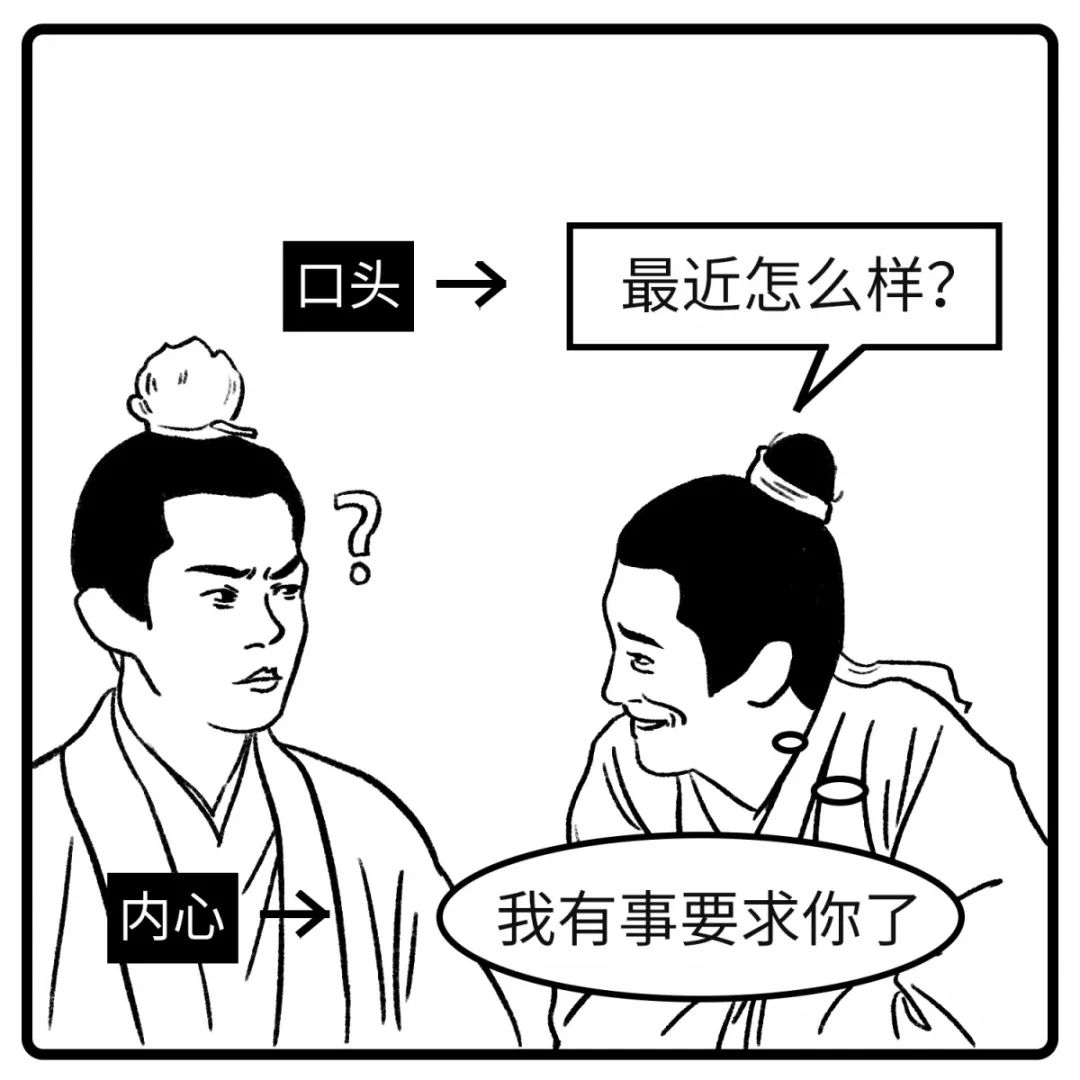15 你有事吗?

=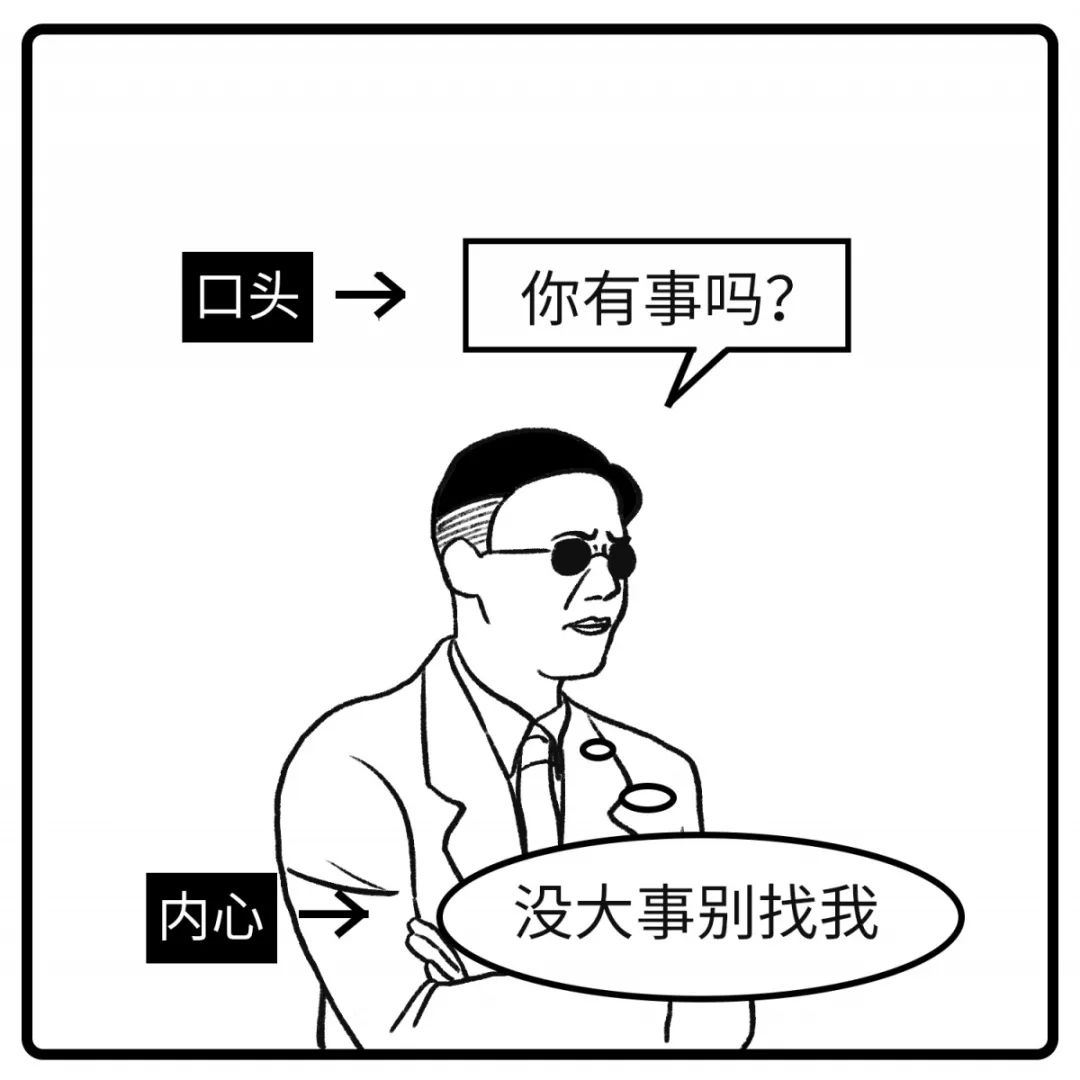16 我不是针对你

=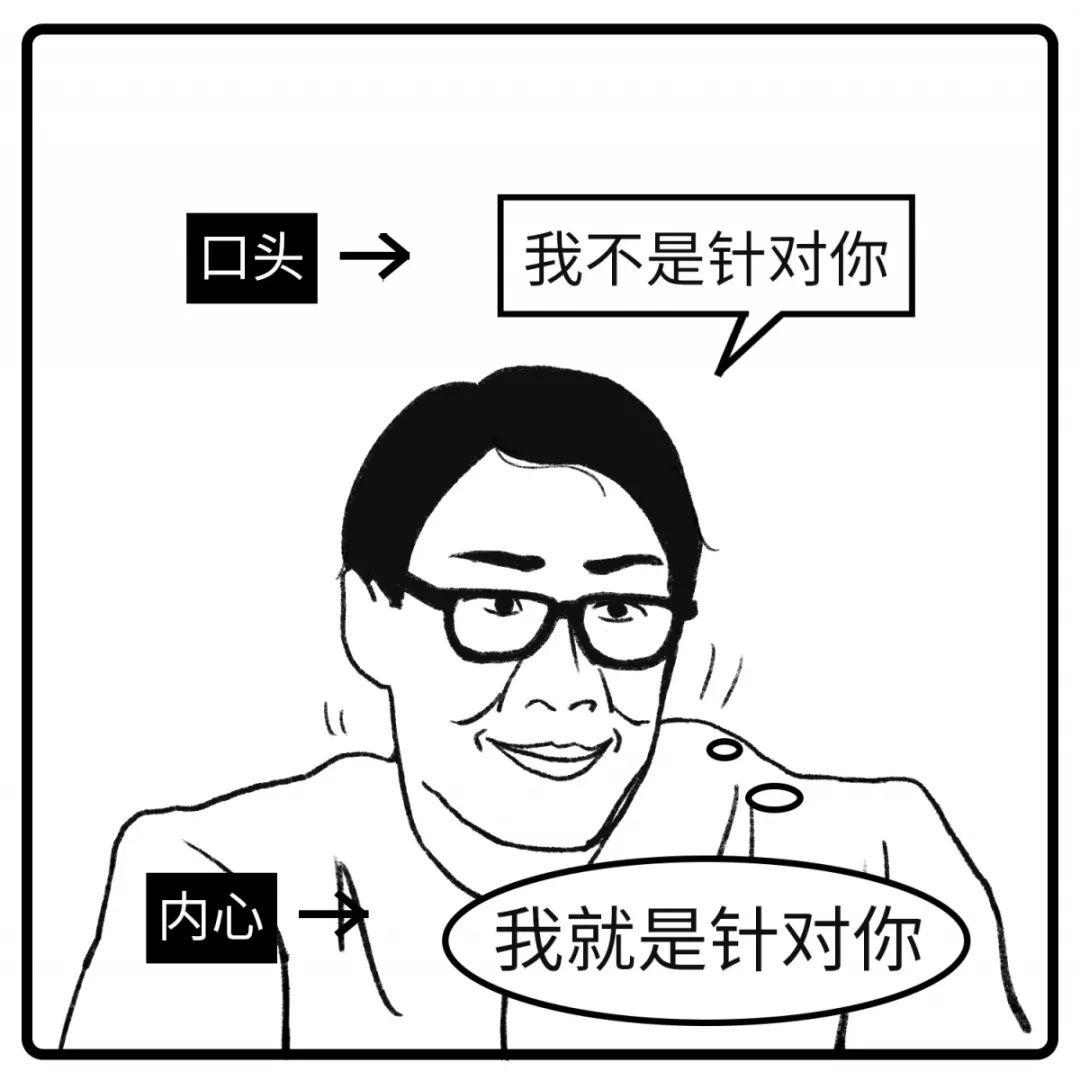17 这不是钱的问题

=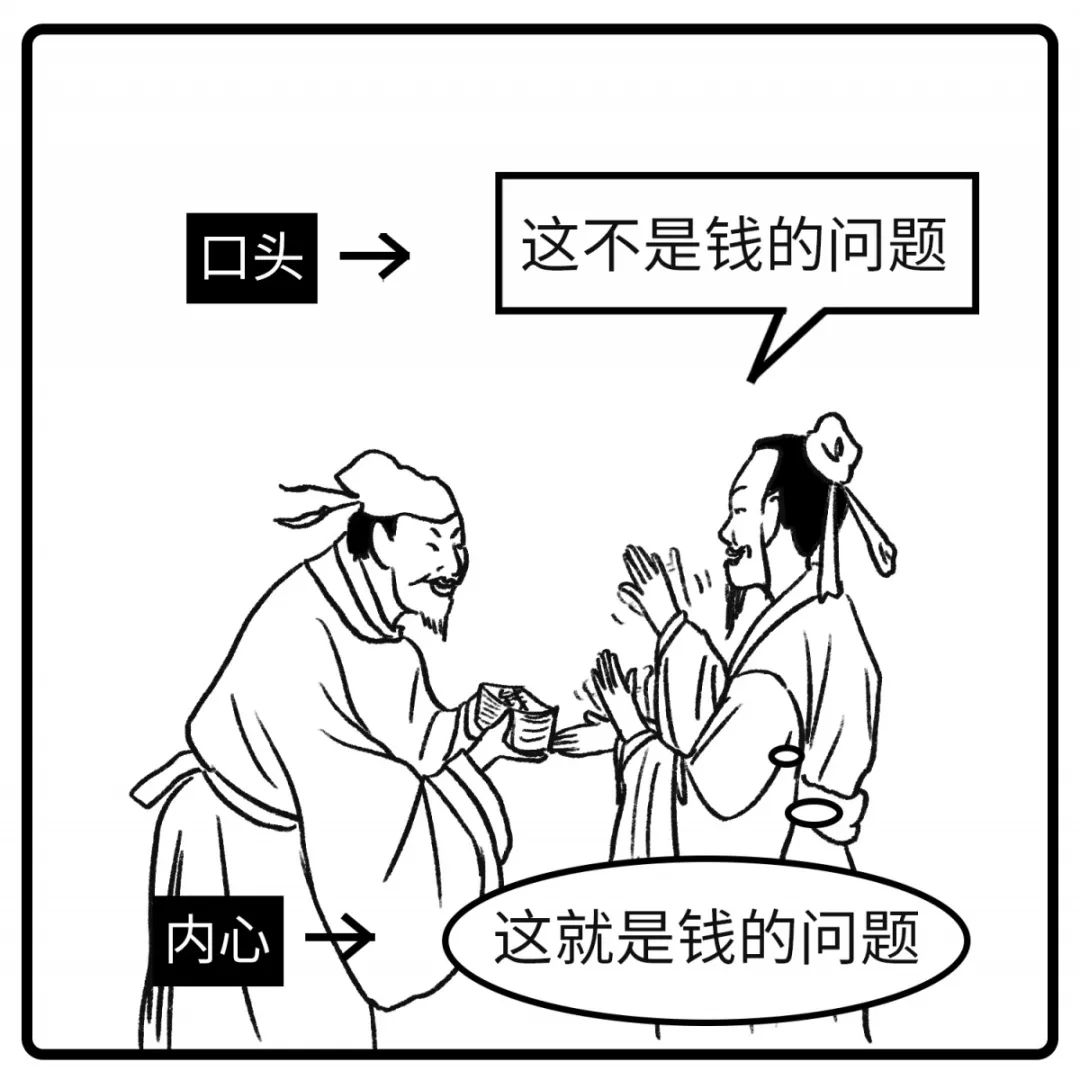18 我尽量吧

=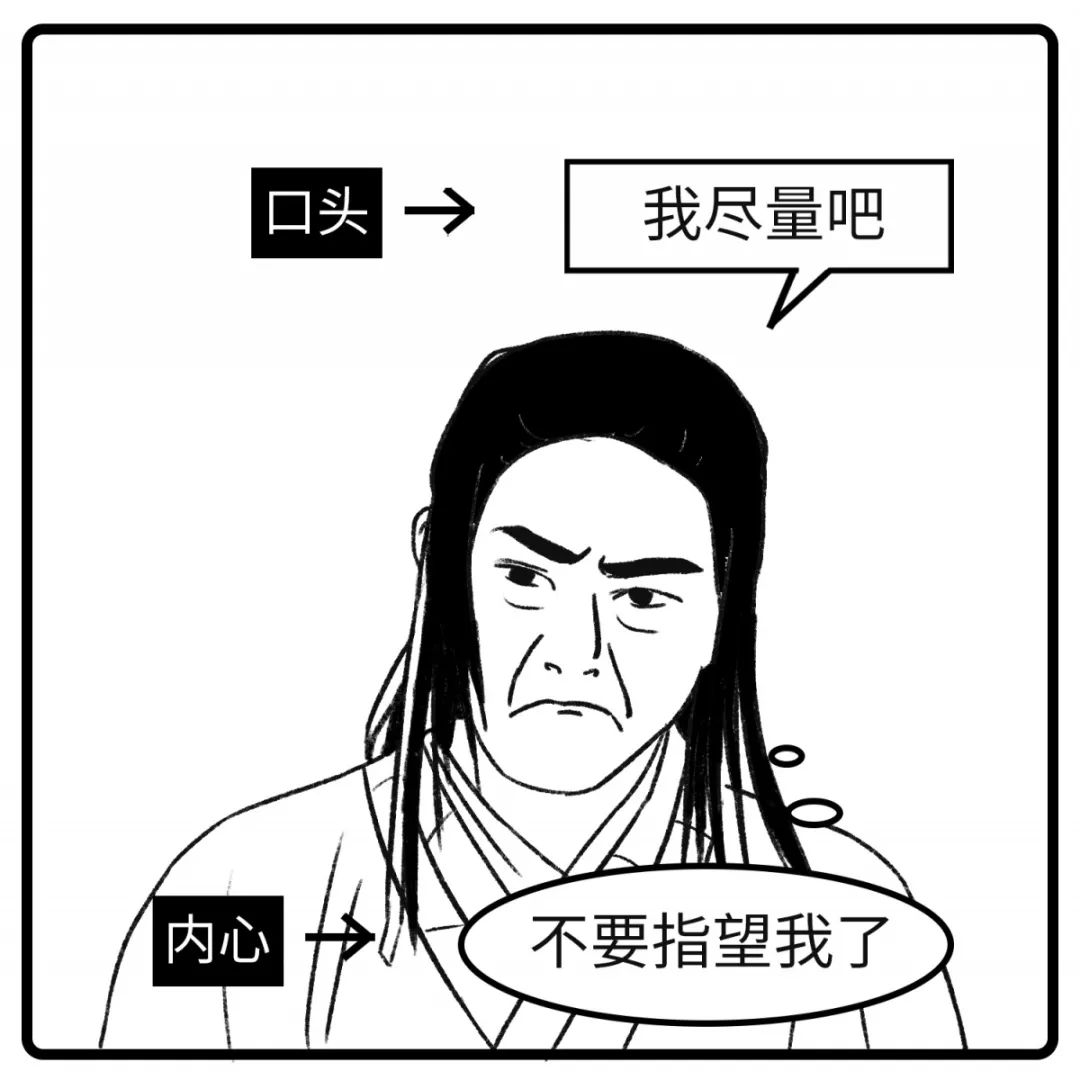19 这事不好办

=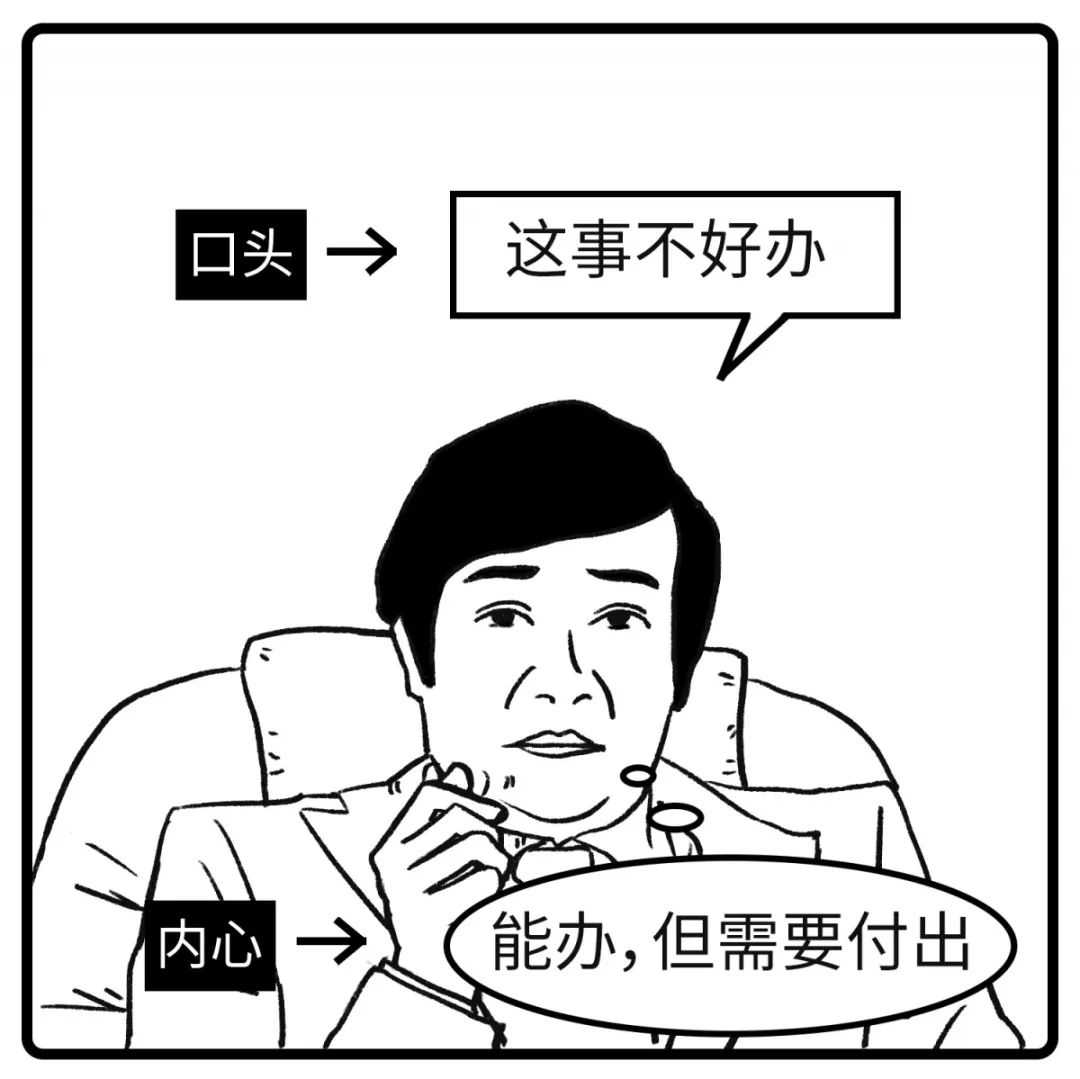-20-

=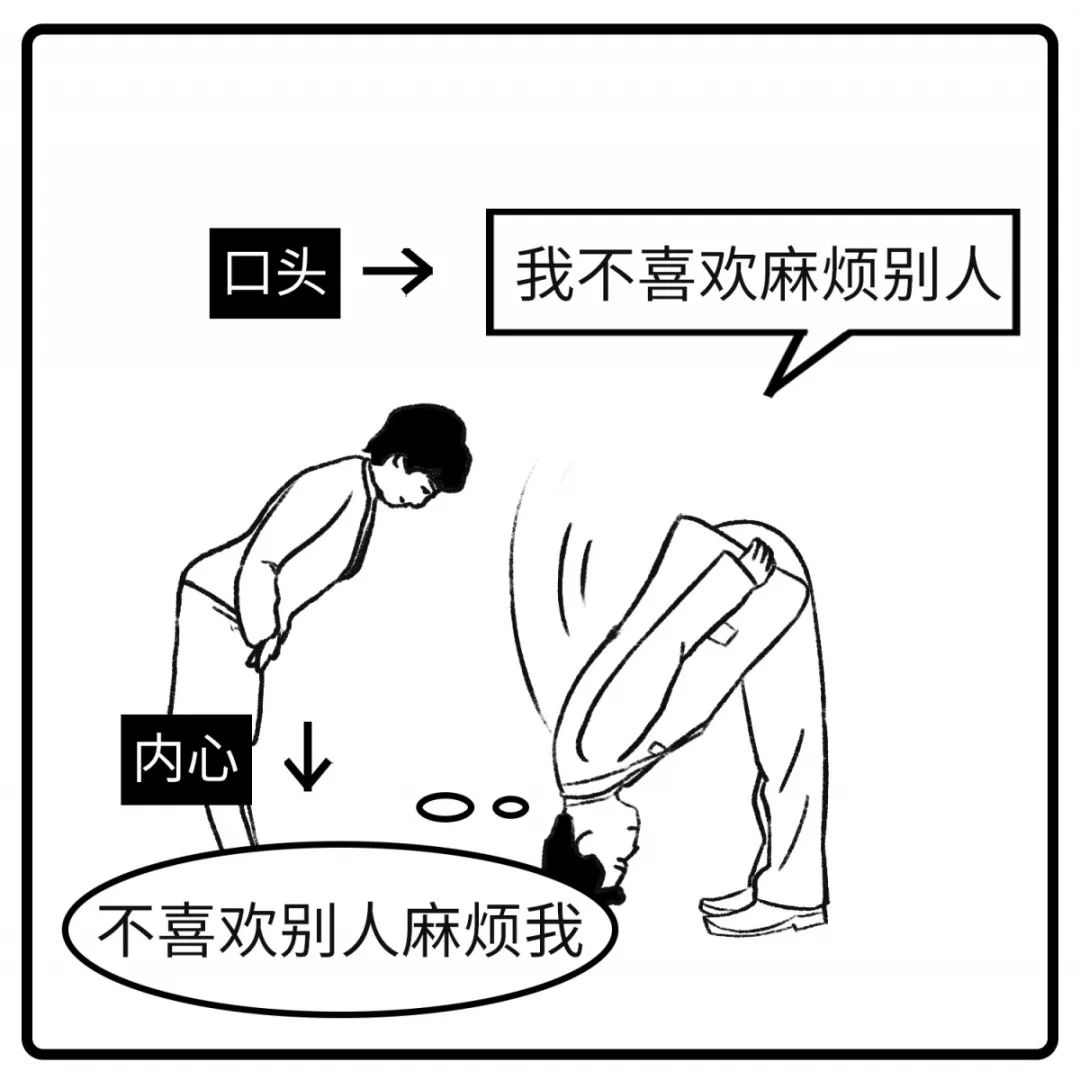21 还好

=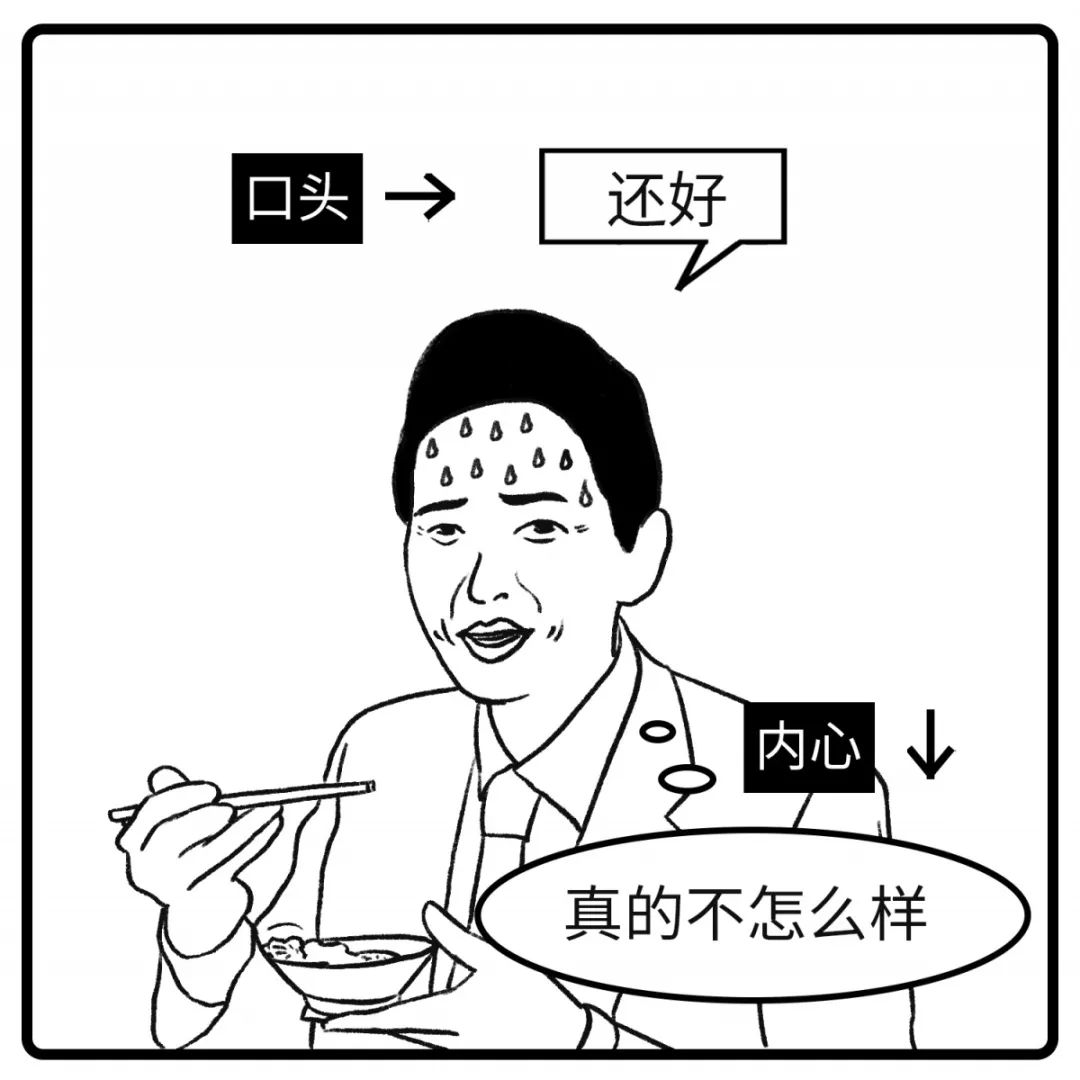22 没关系，我没事

=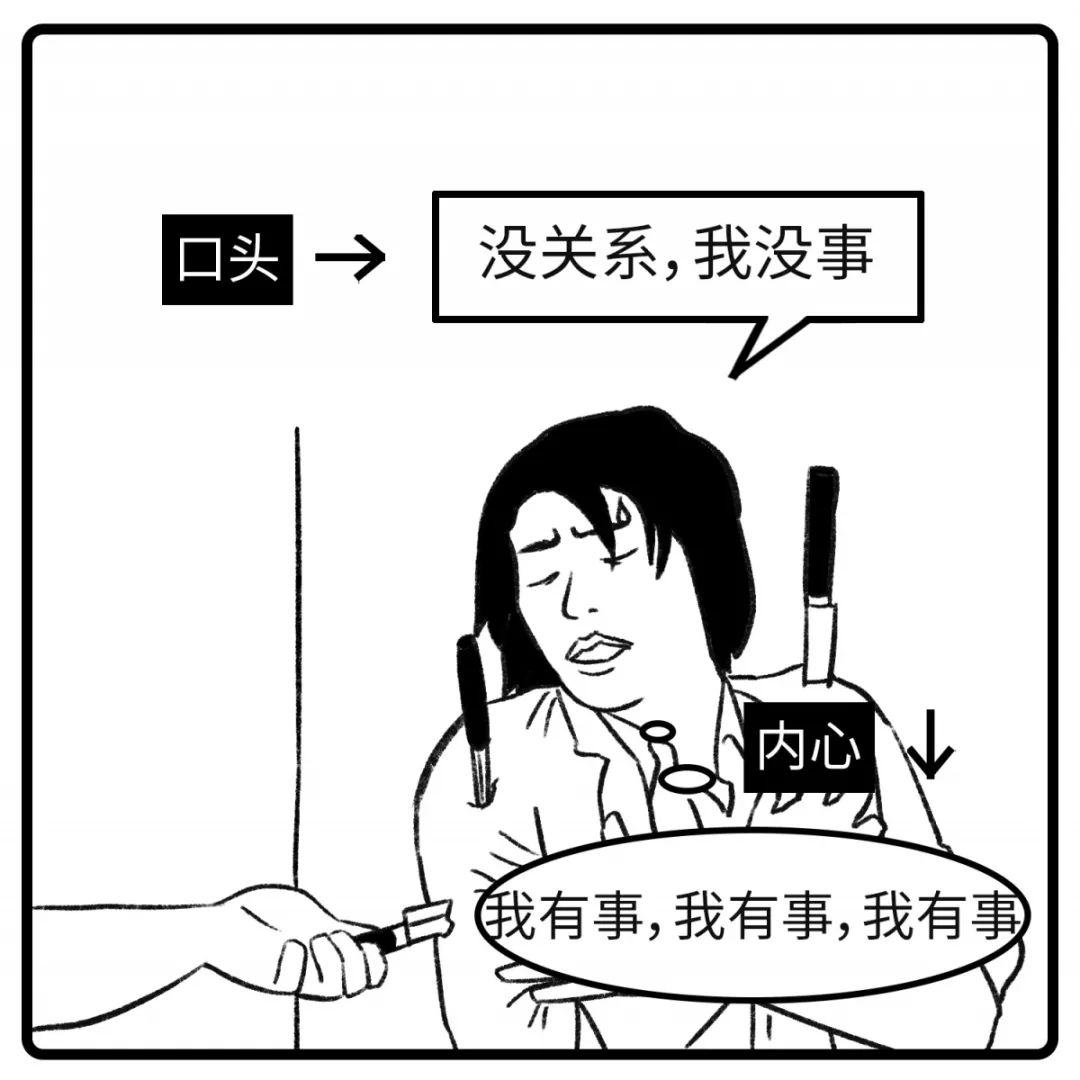23 哦，随便你

=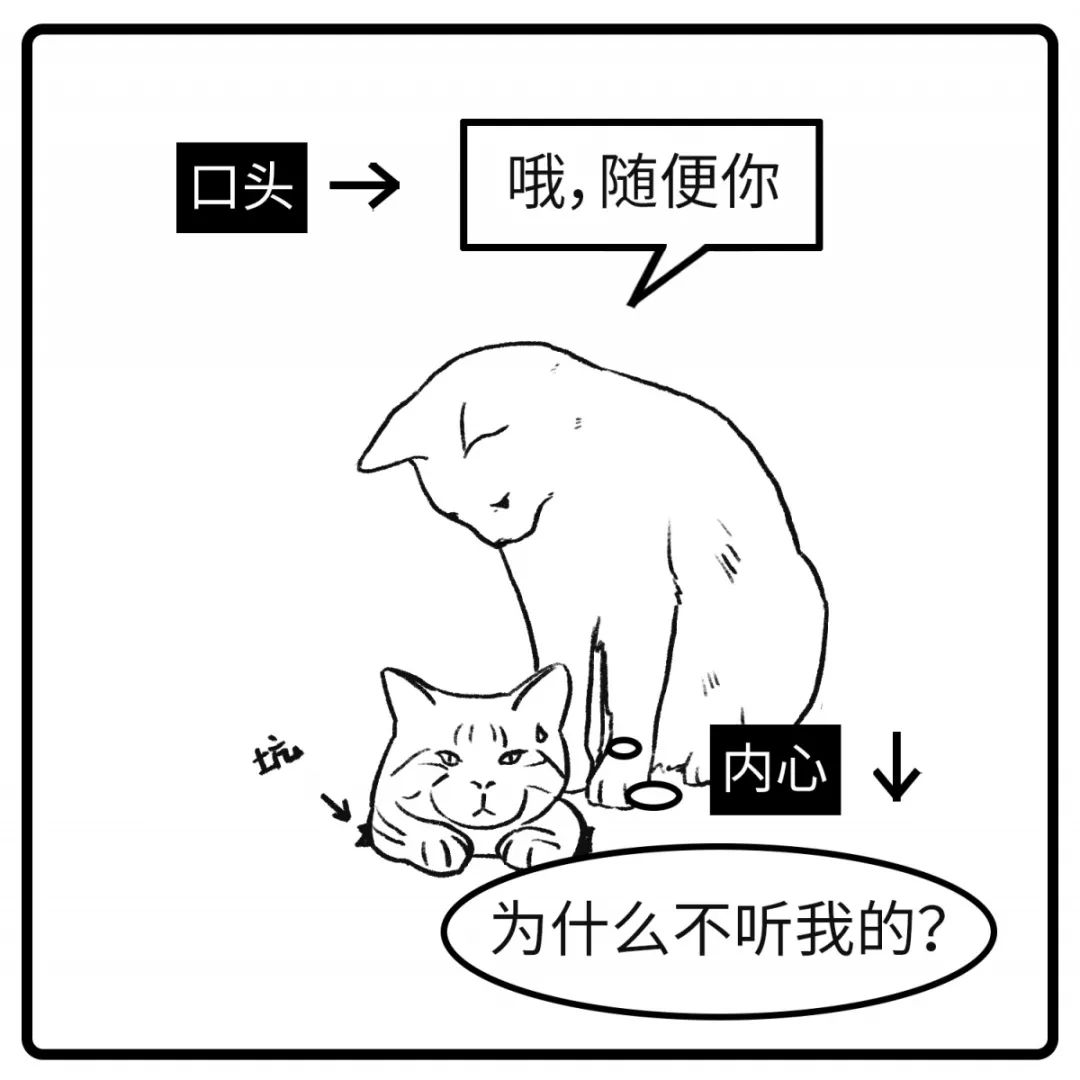24 那好吧，不打扰你了

=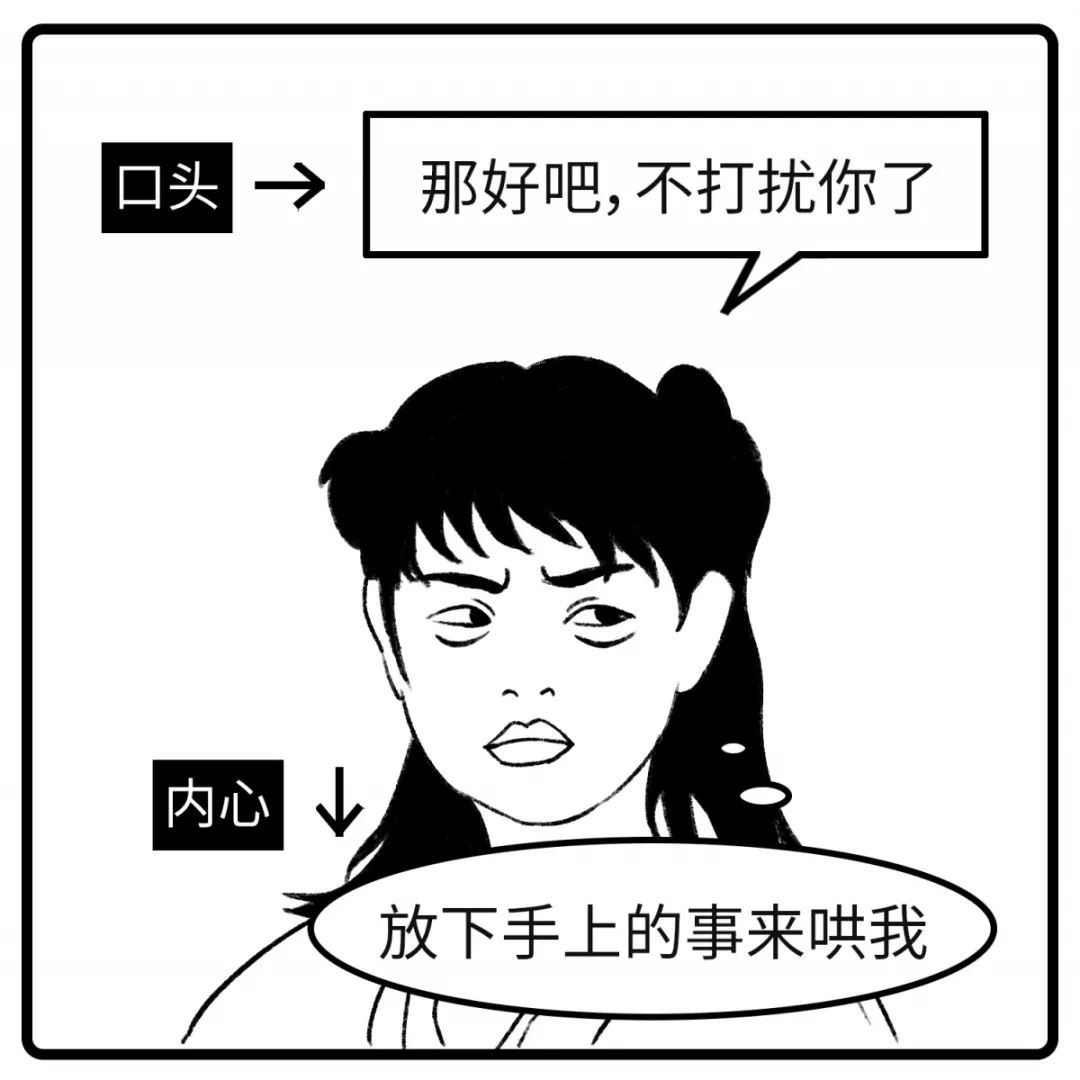25 再也不想看见你了

=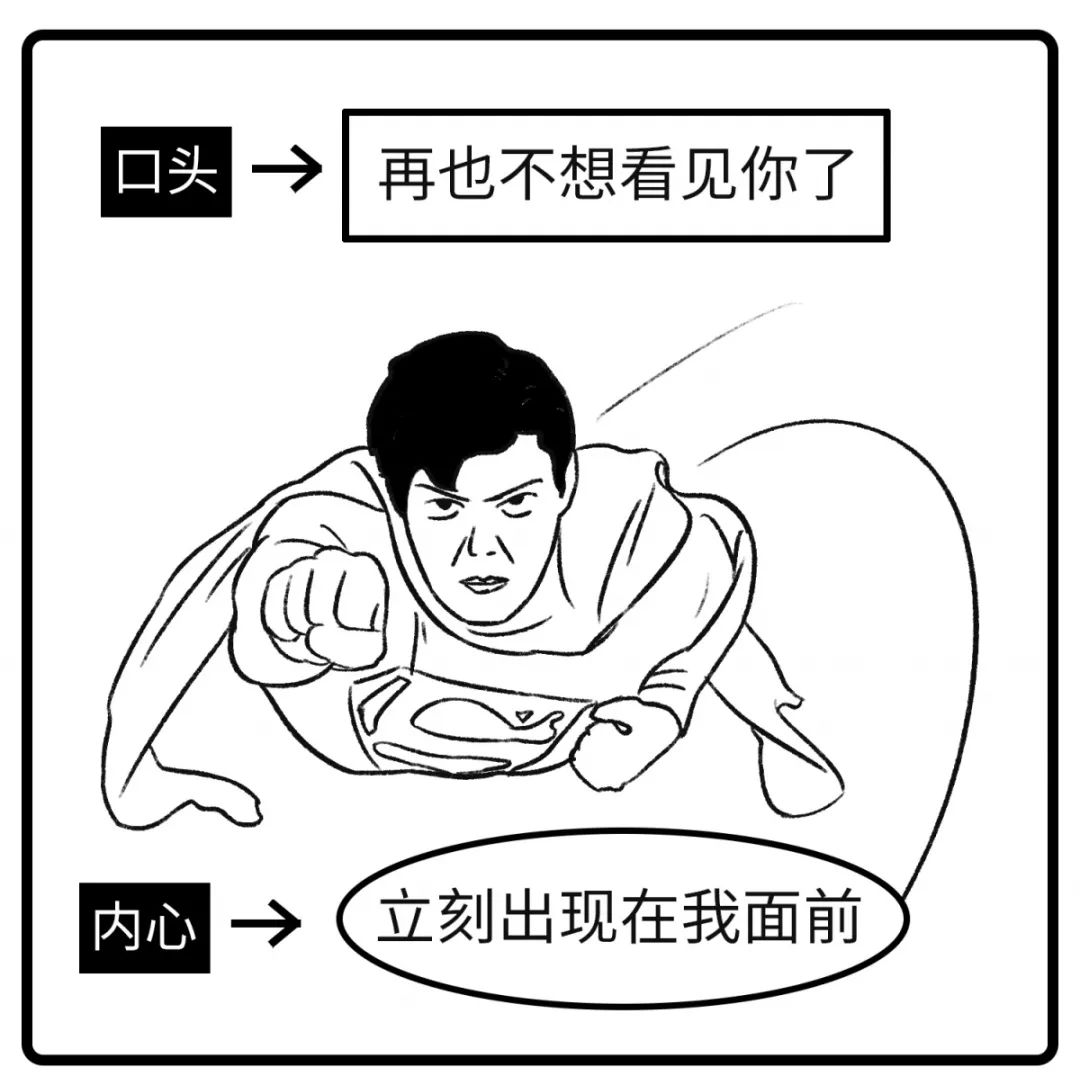26 这件衣服太贵了

=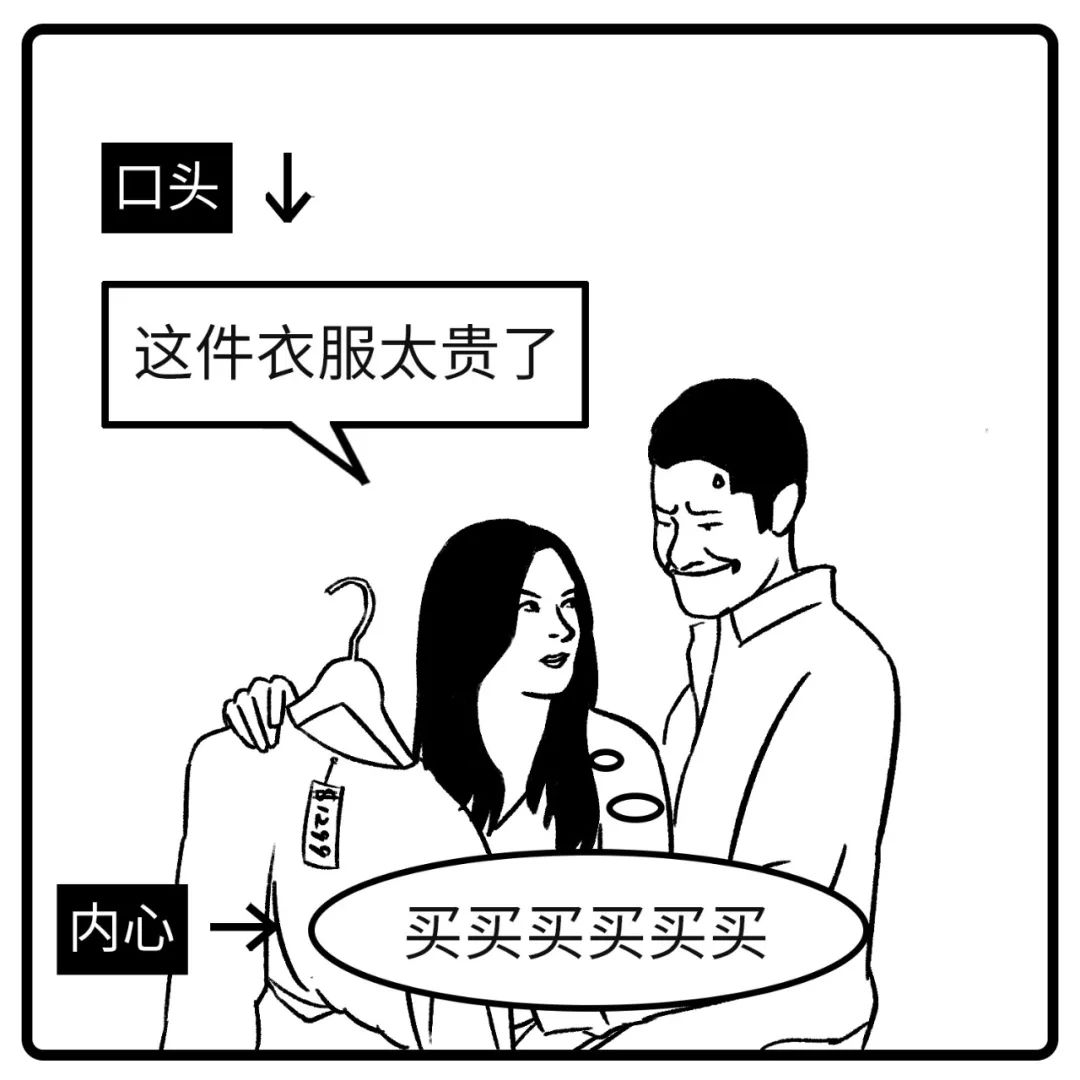27 你比照片好看

=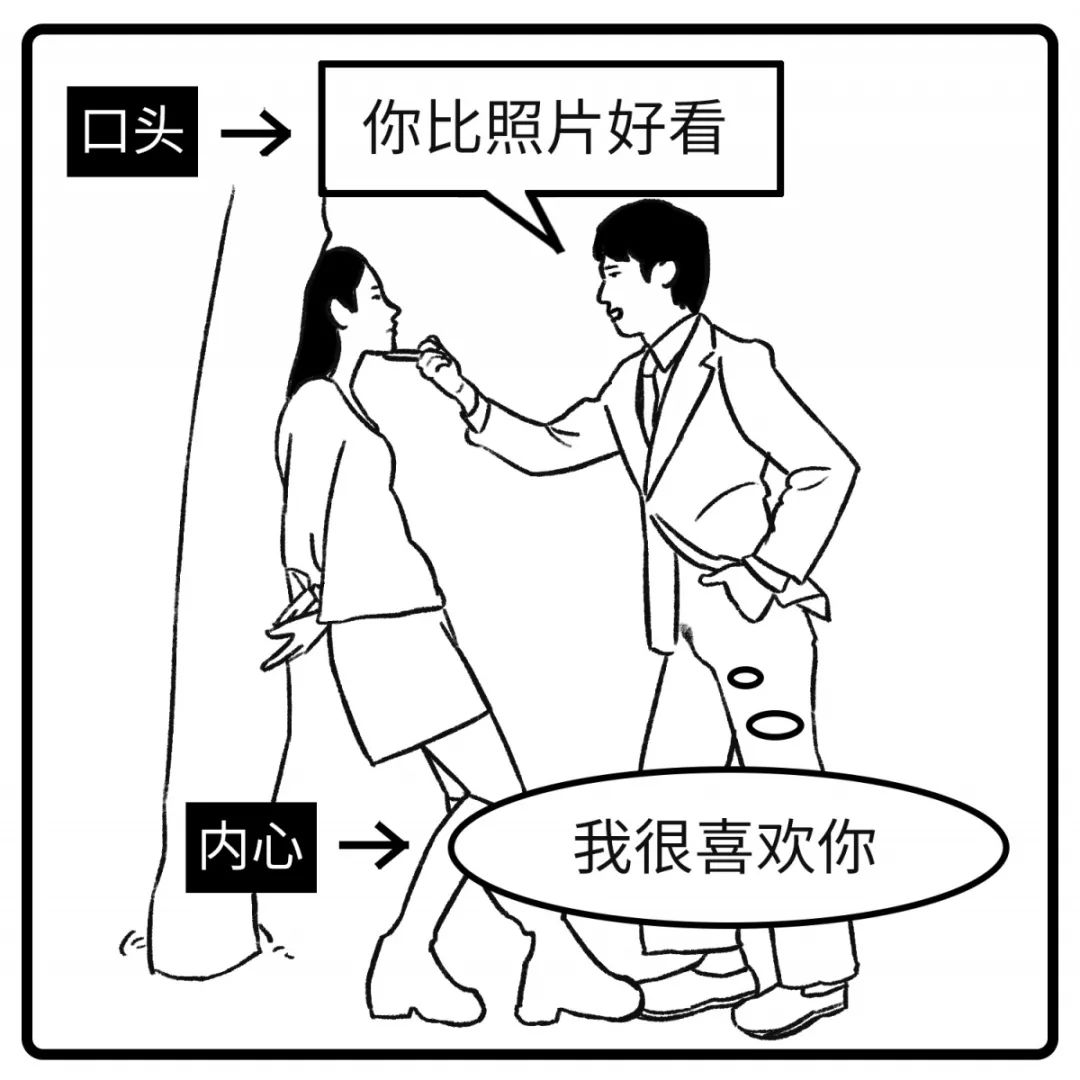28 要不我送你回家吧

=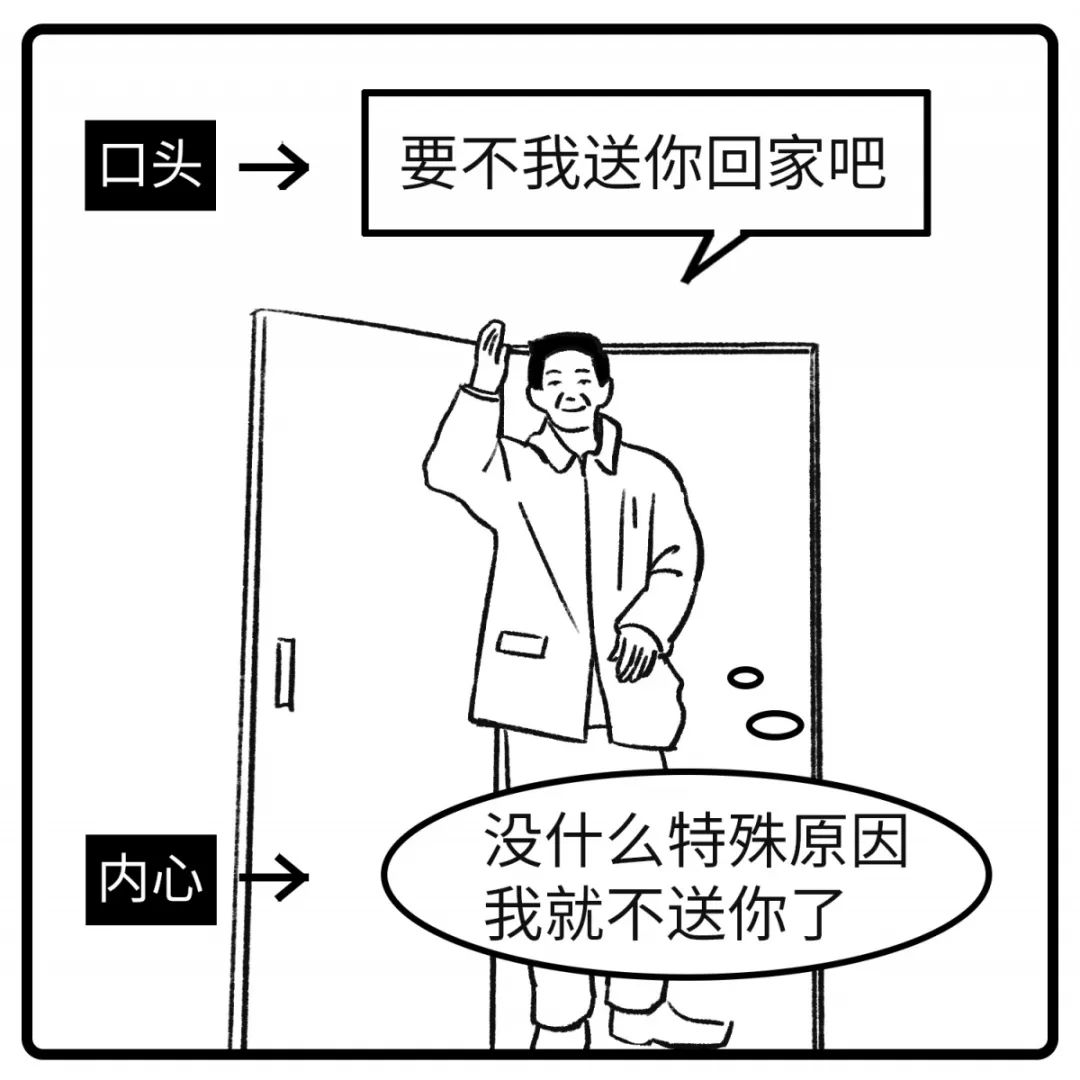29 我送你回家吧

=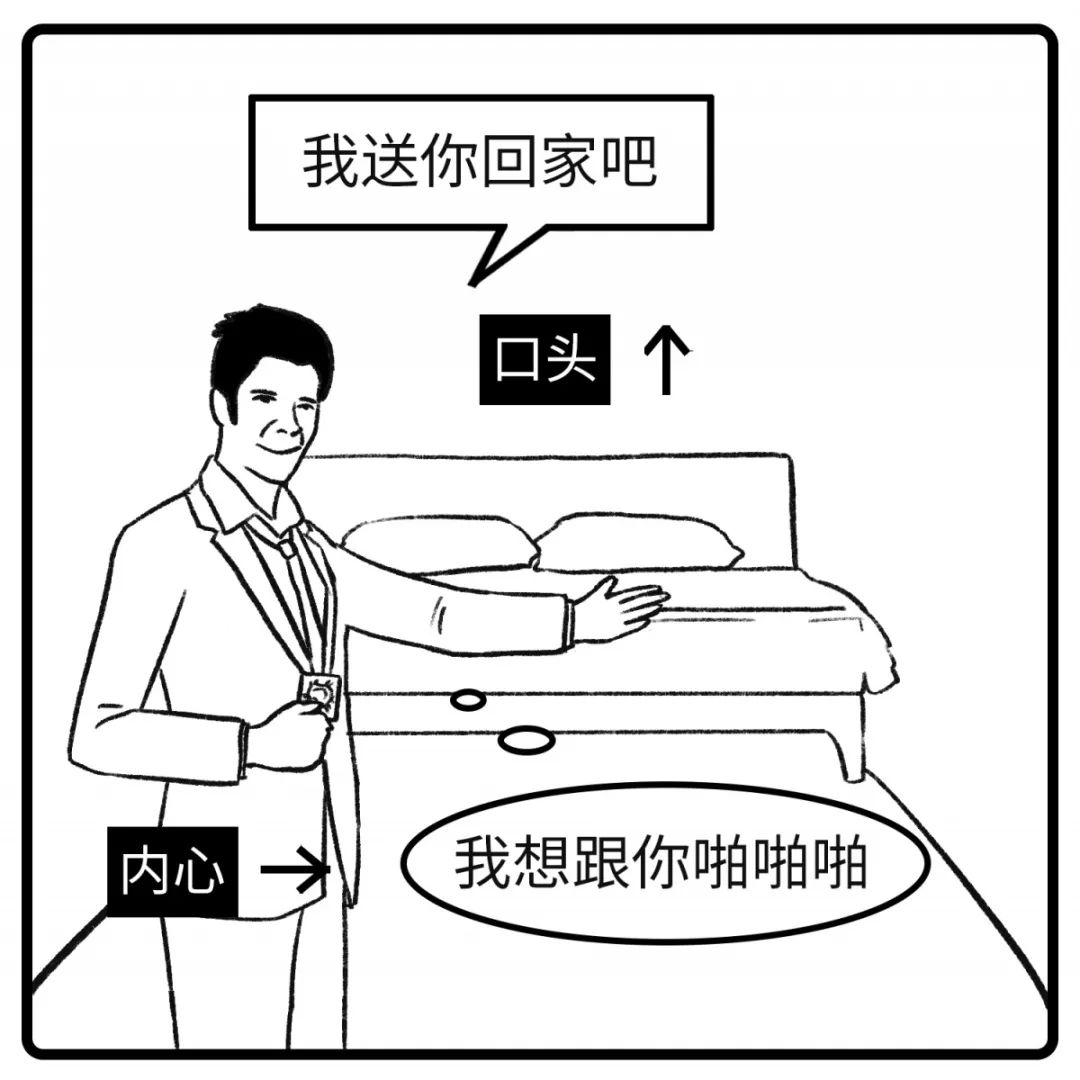30 改天请你吃饭

=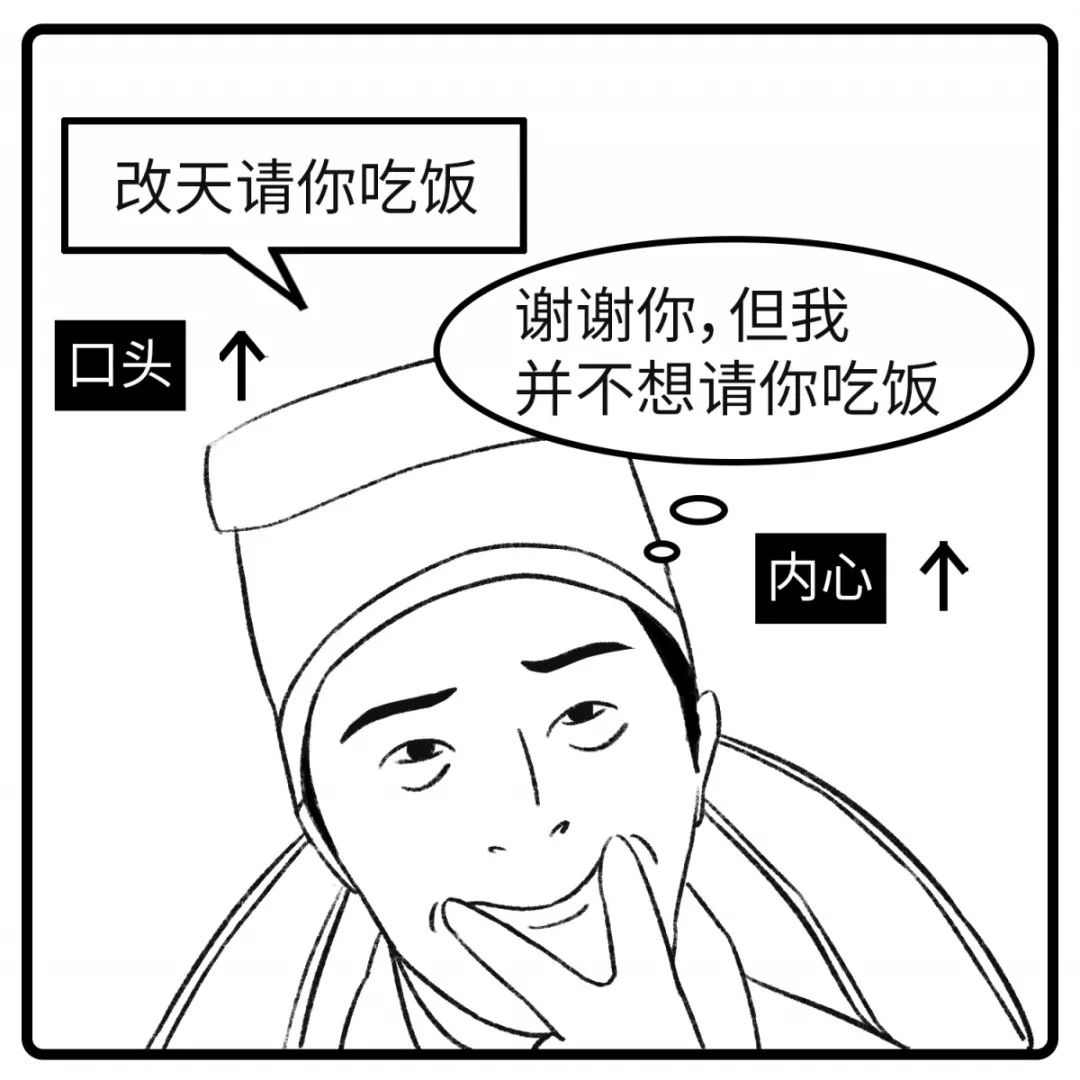31 其实我无所谓的

=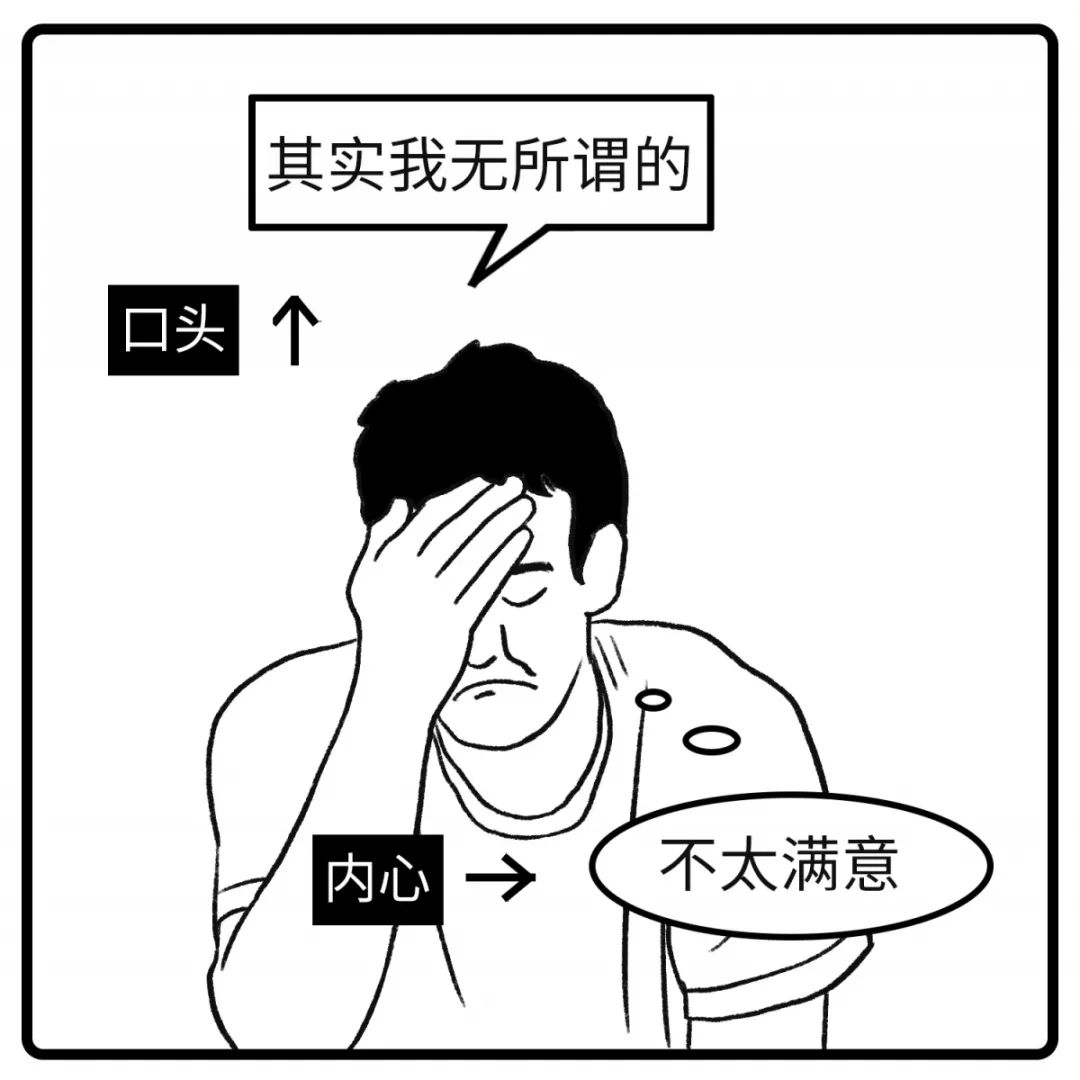32 领导对你不错啊

=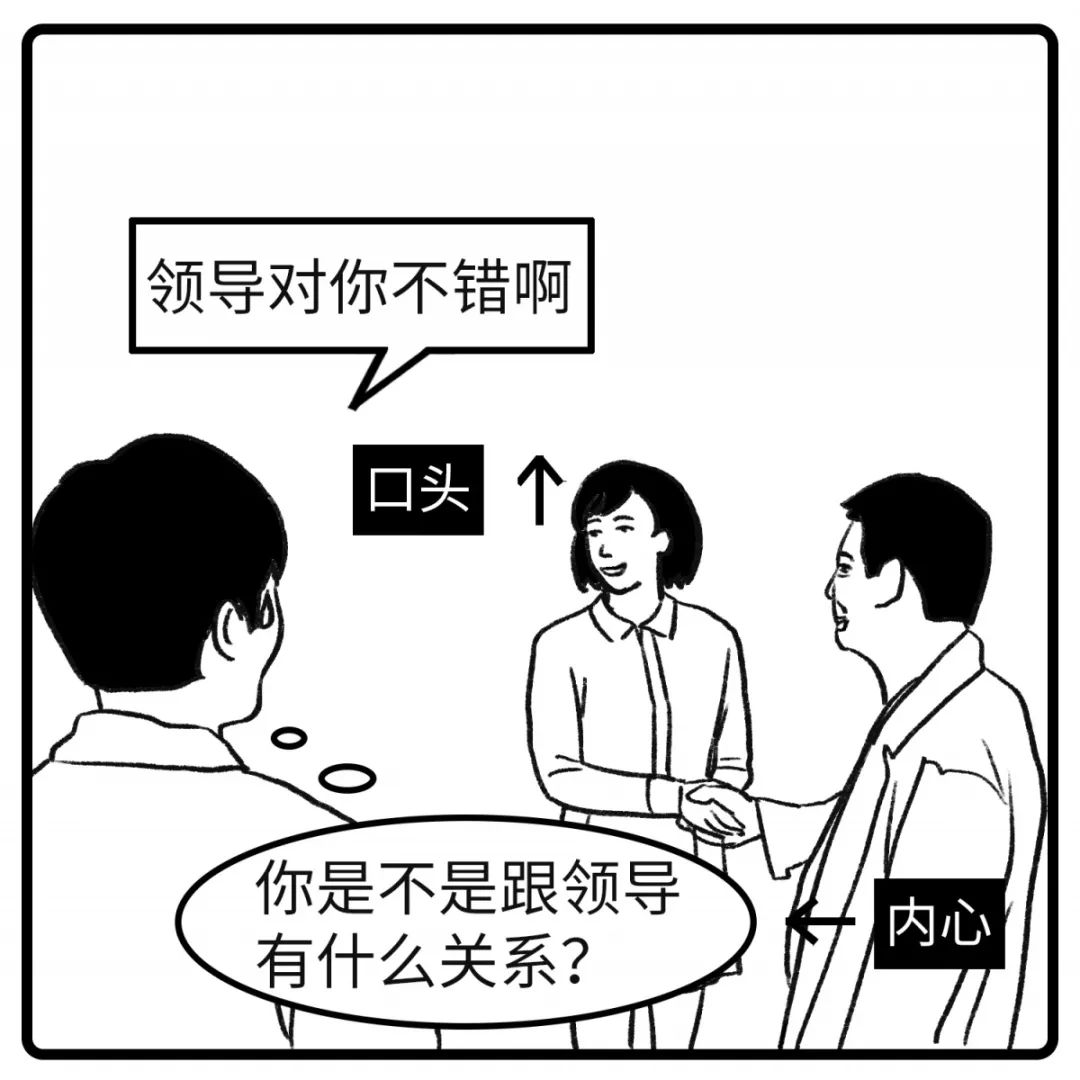33 我们再考虑一下

=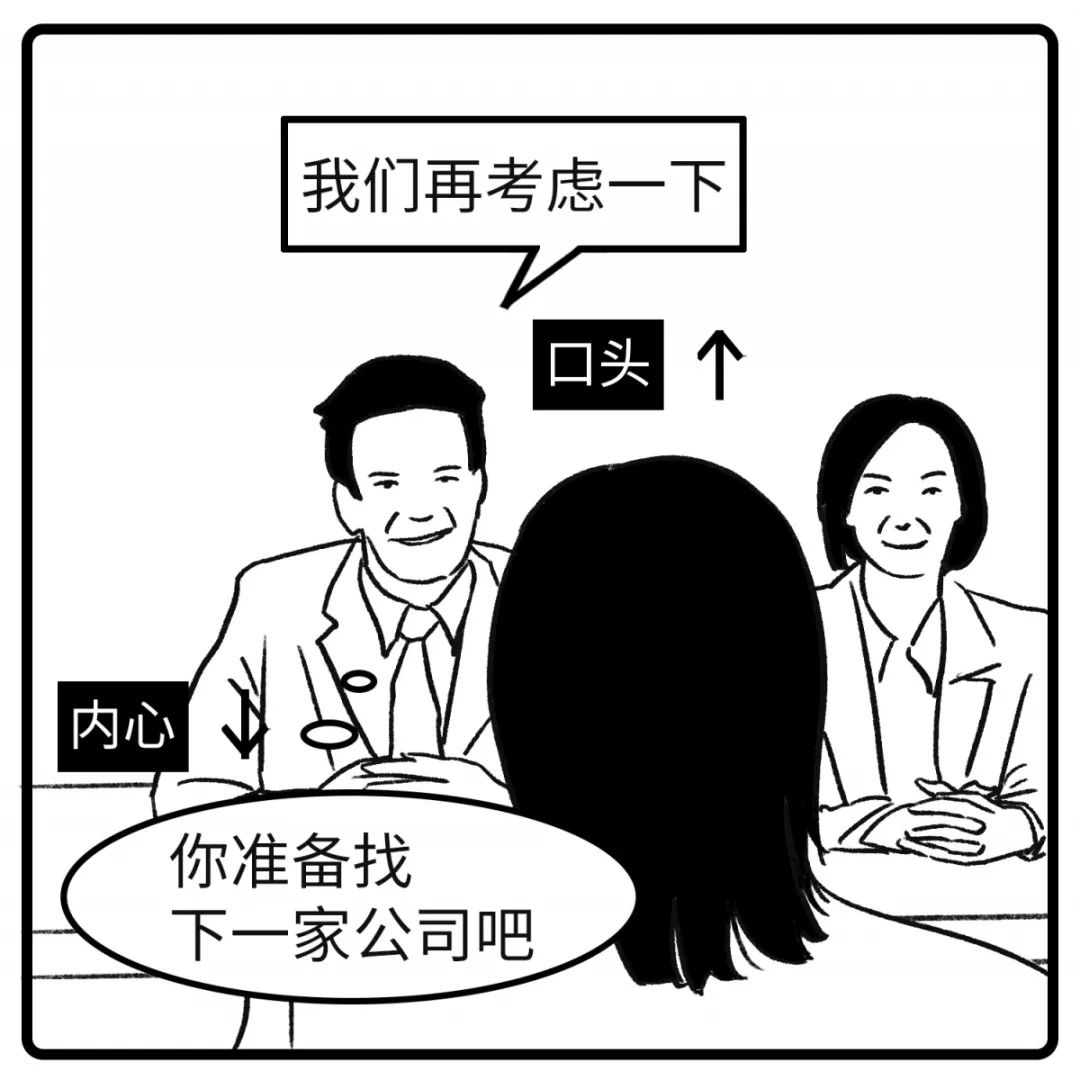34 最近家里是不是有什么问题？

=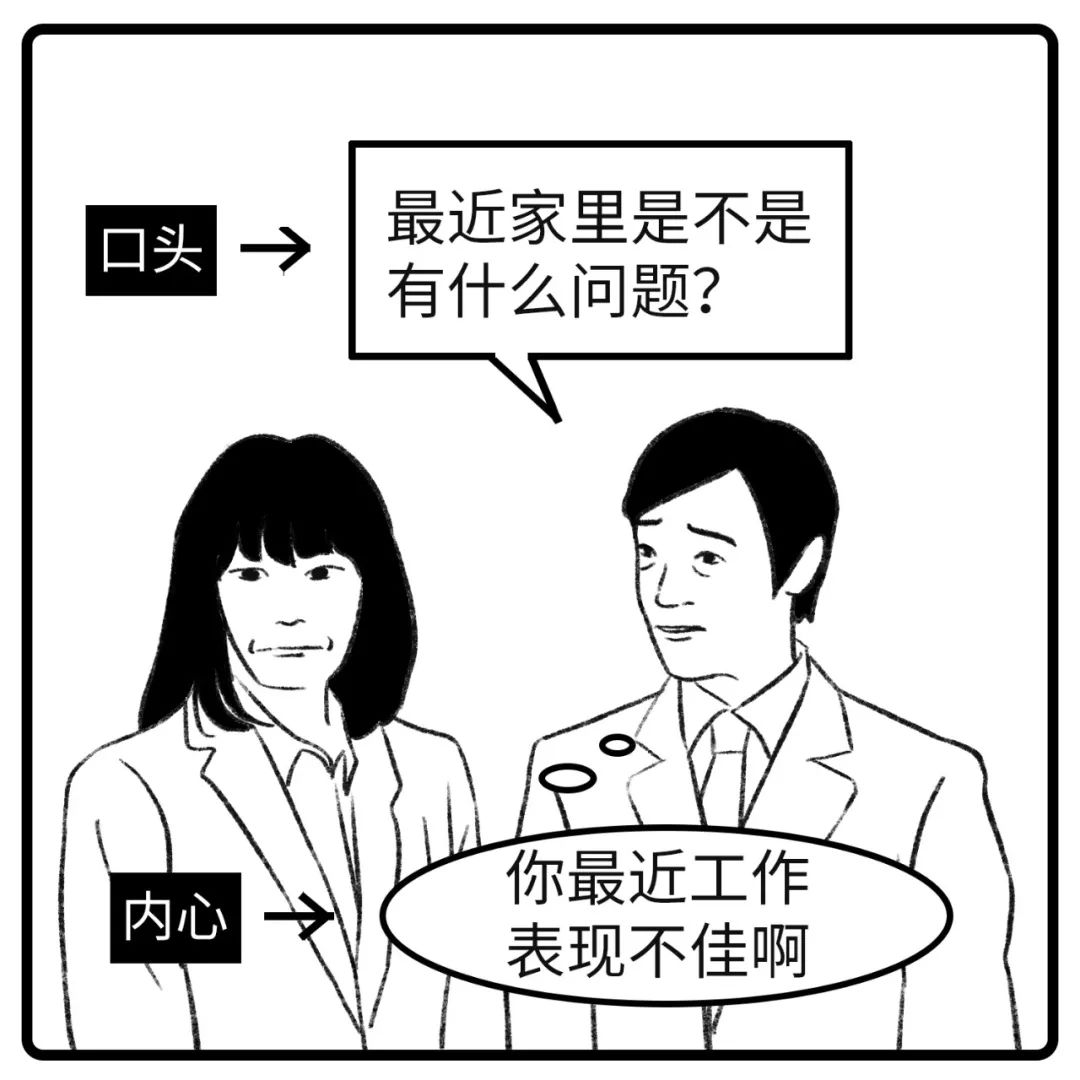35 原则上不行

=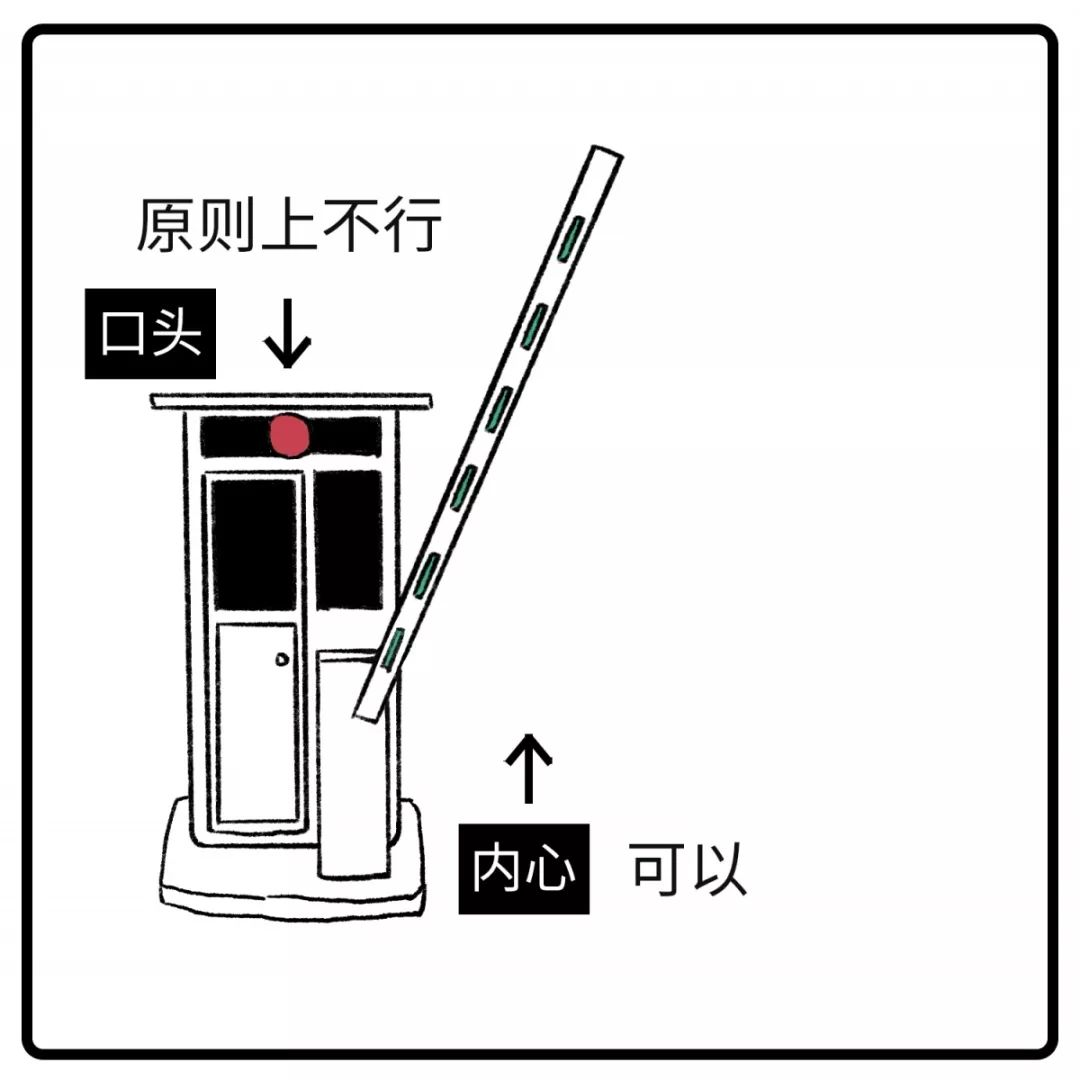36 原则上可以

=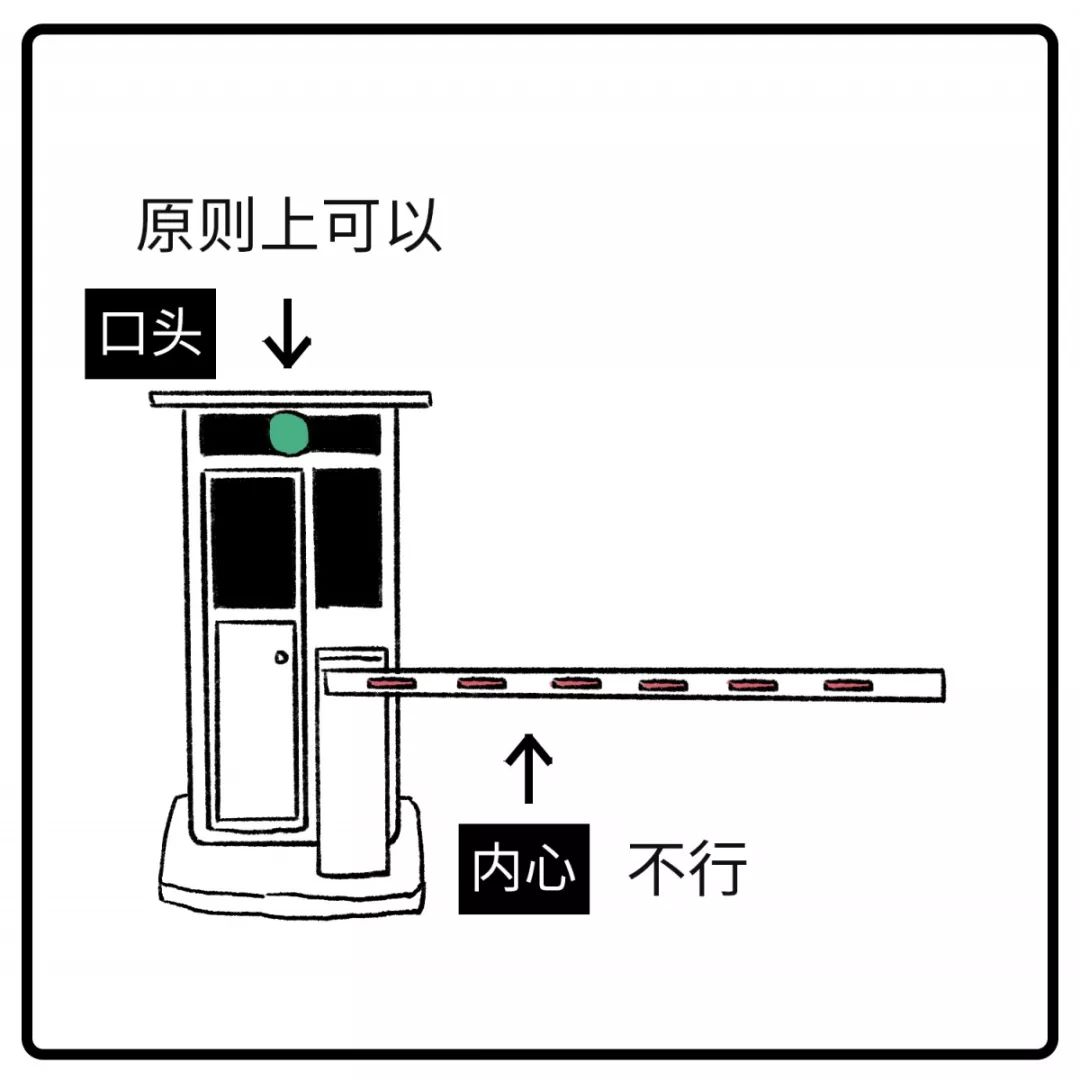37 说句公道话

=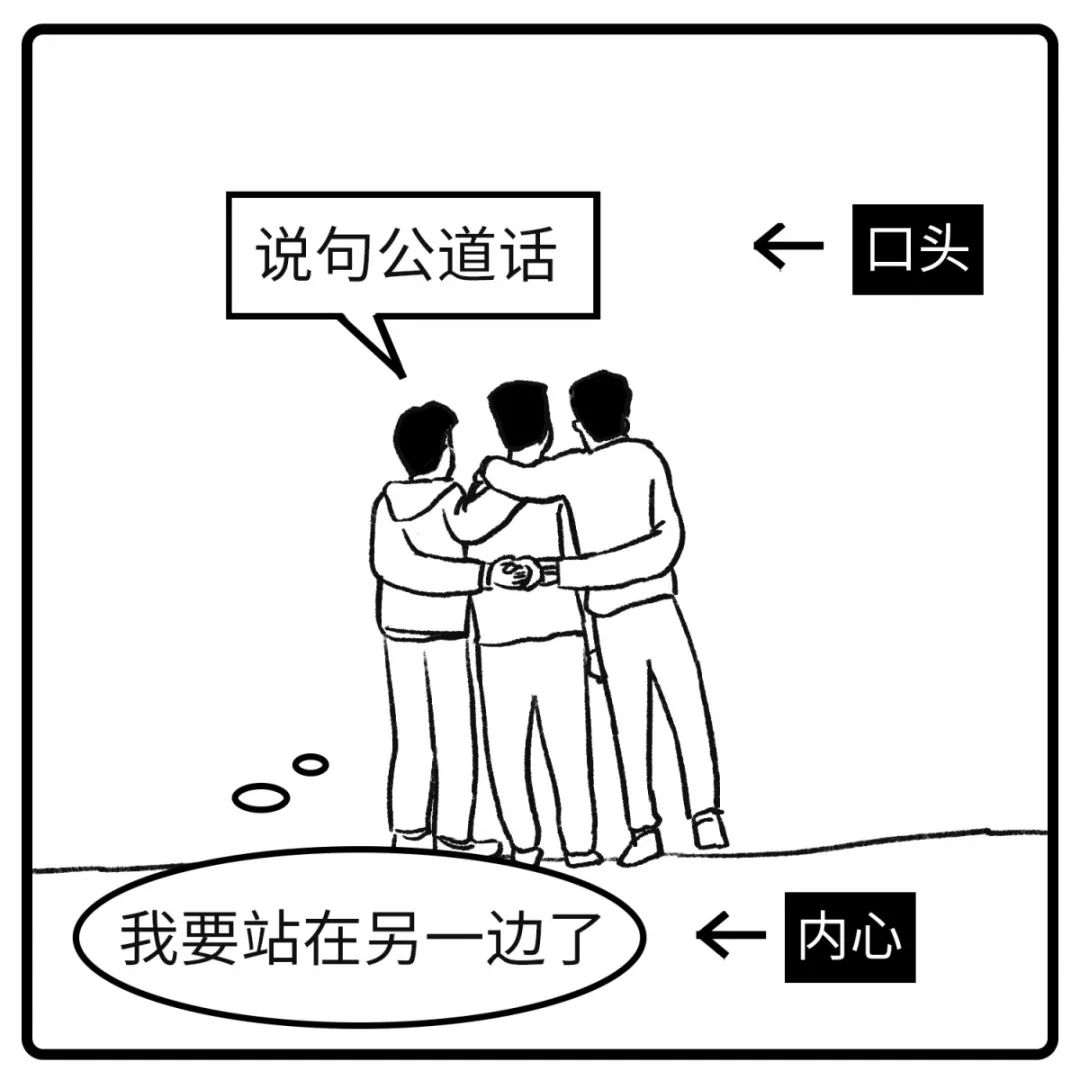38 我手机要没电了

=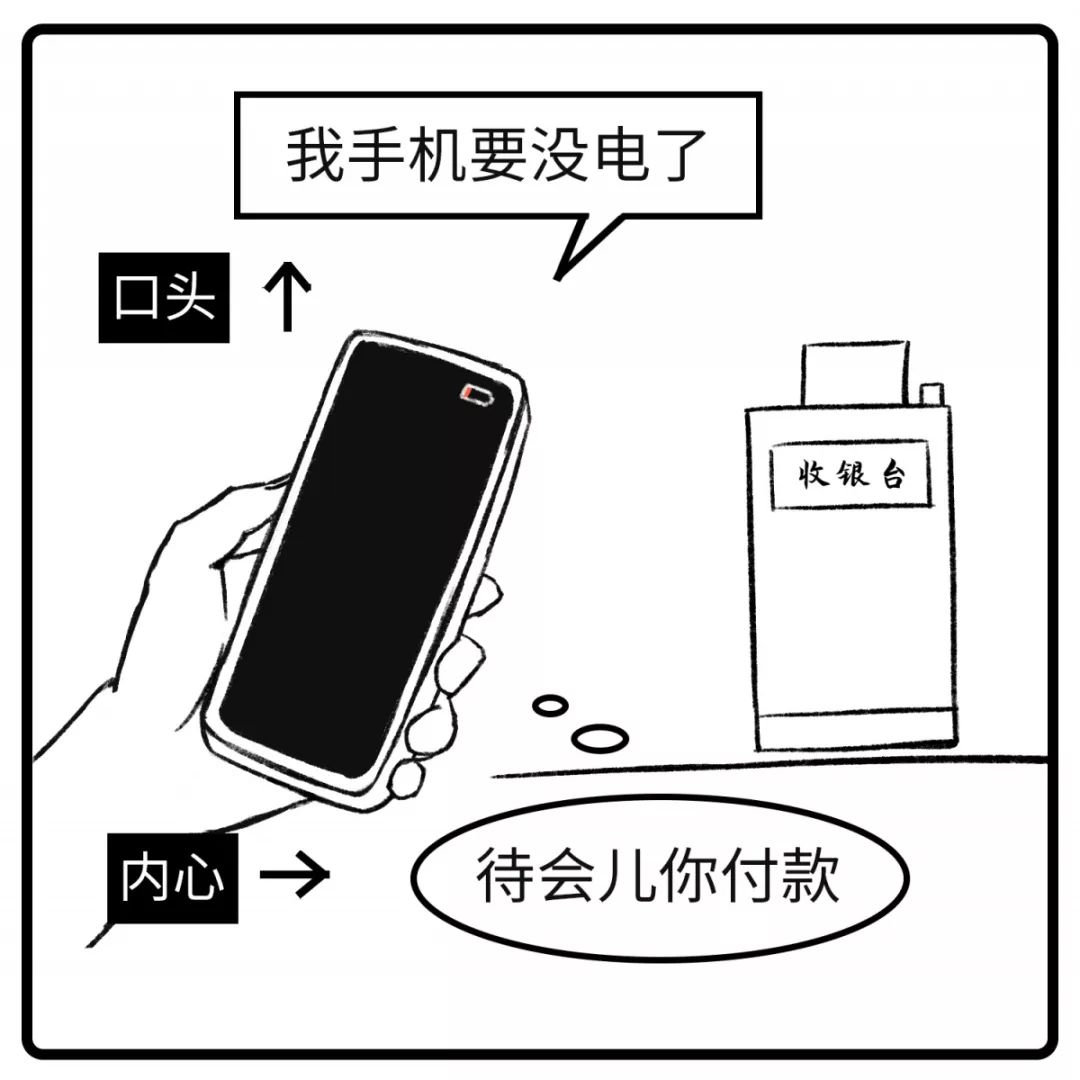39 哈哈

=40 我们现在去XX，你要一起吗

=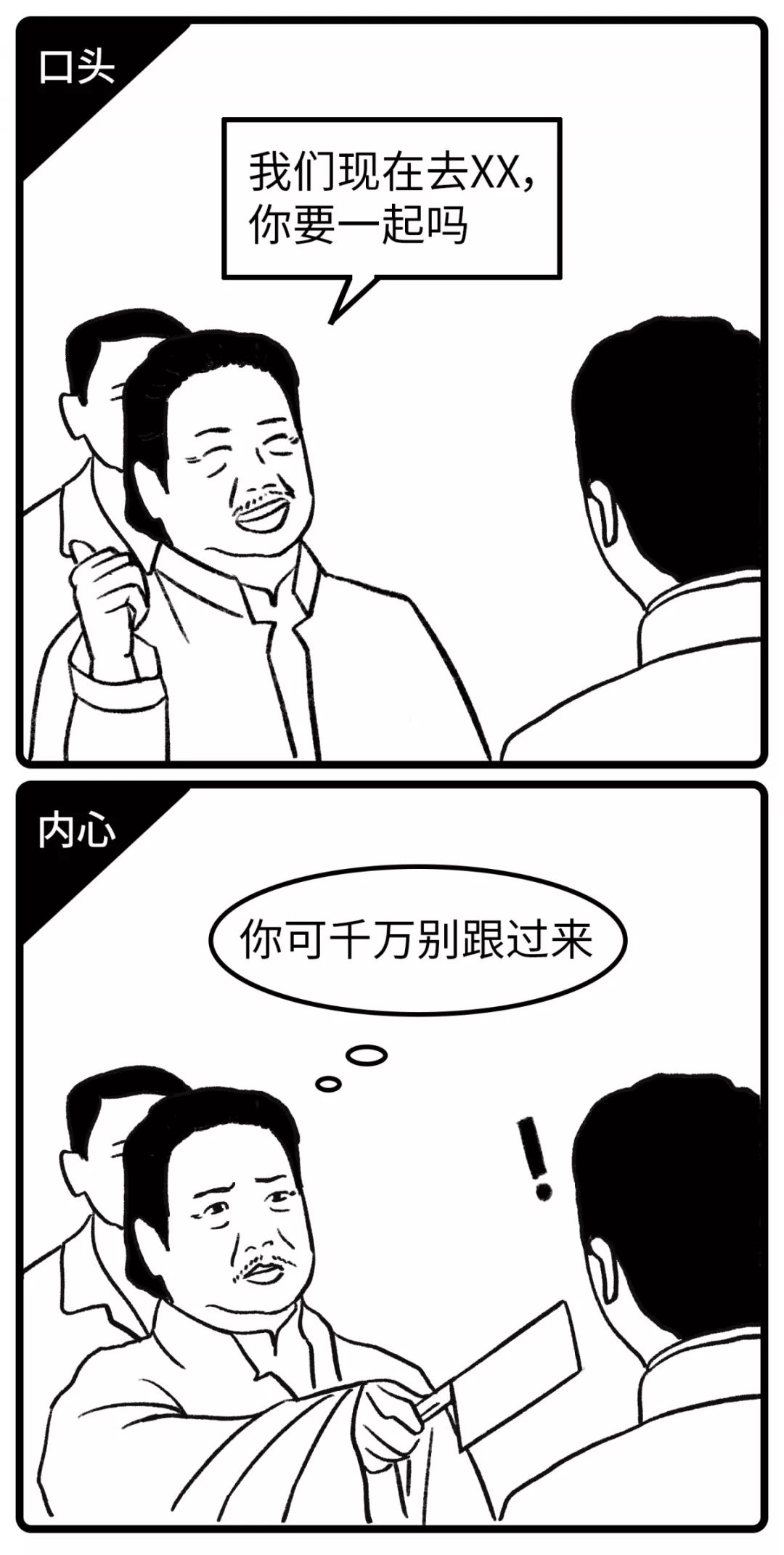41 我再考虑考虑

=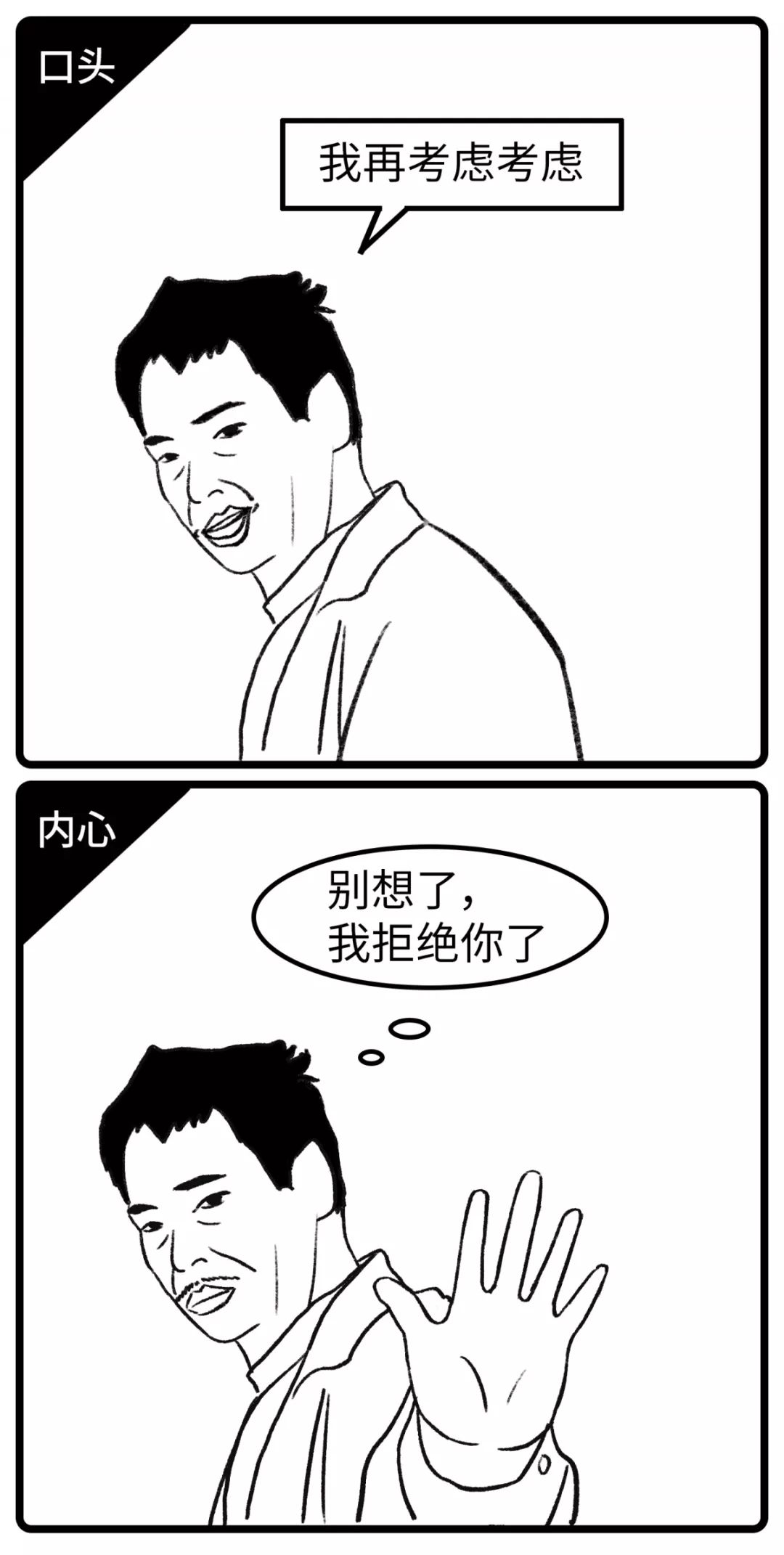42 我没生气

=43 这事儿也不全赖你

=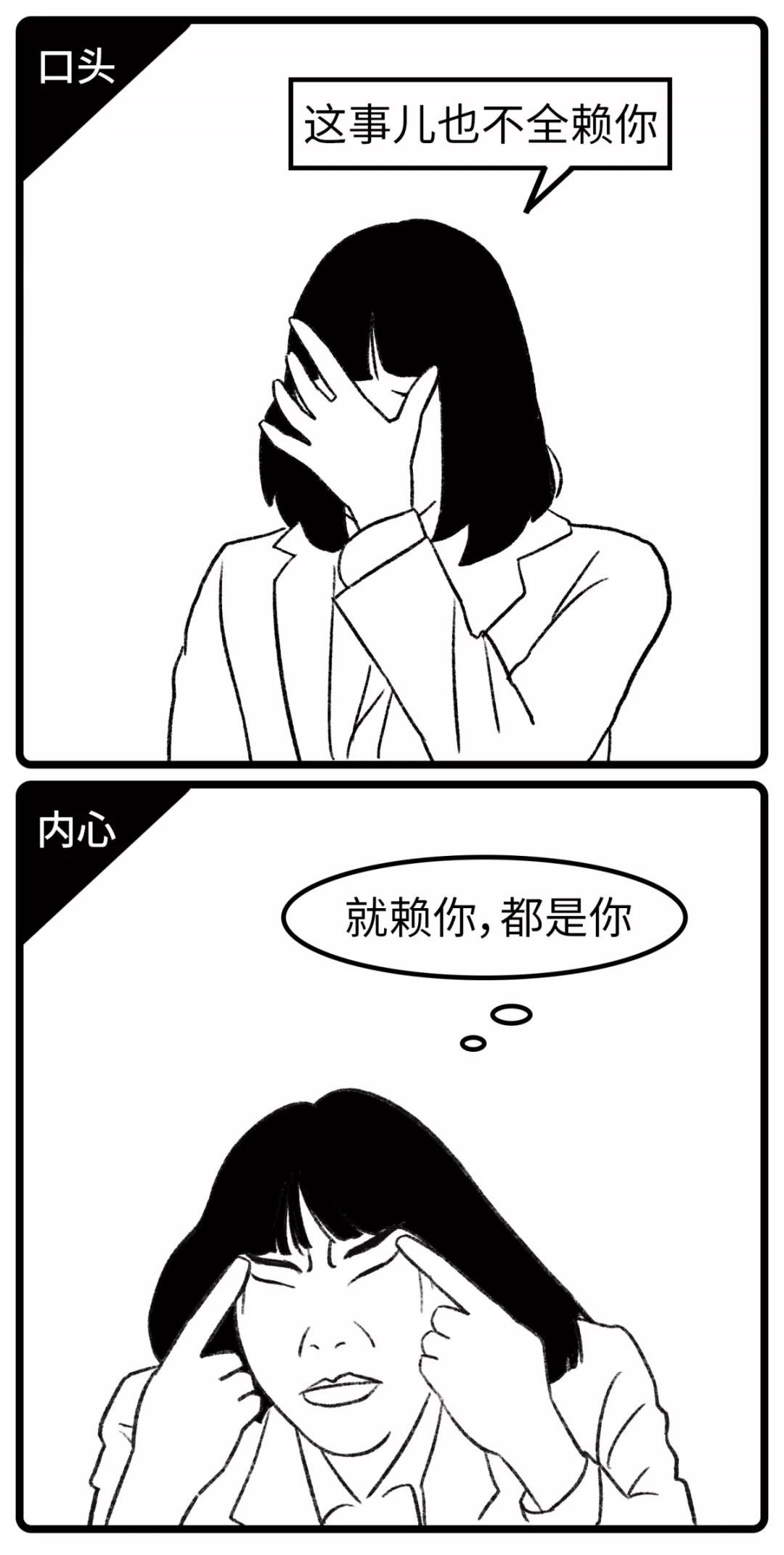44 你喜欢怎么做就怎么做吧

=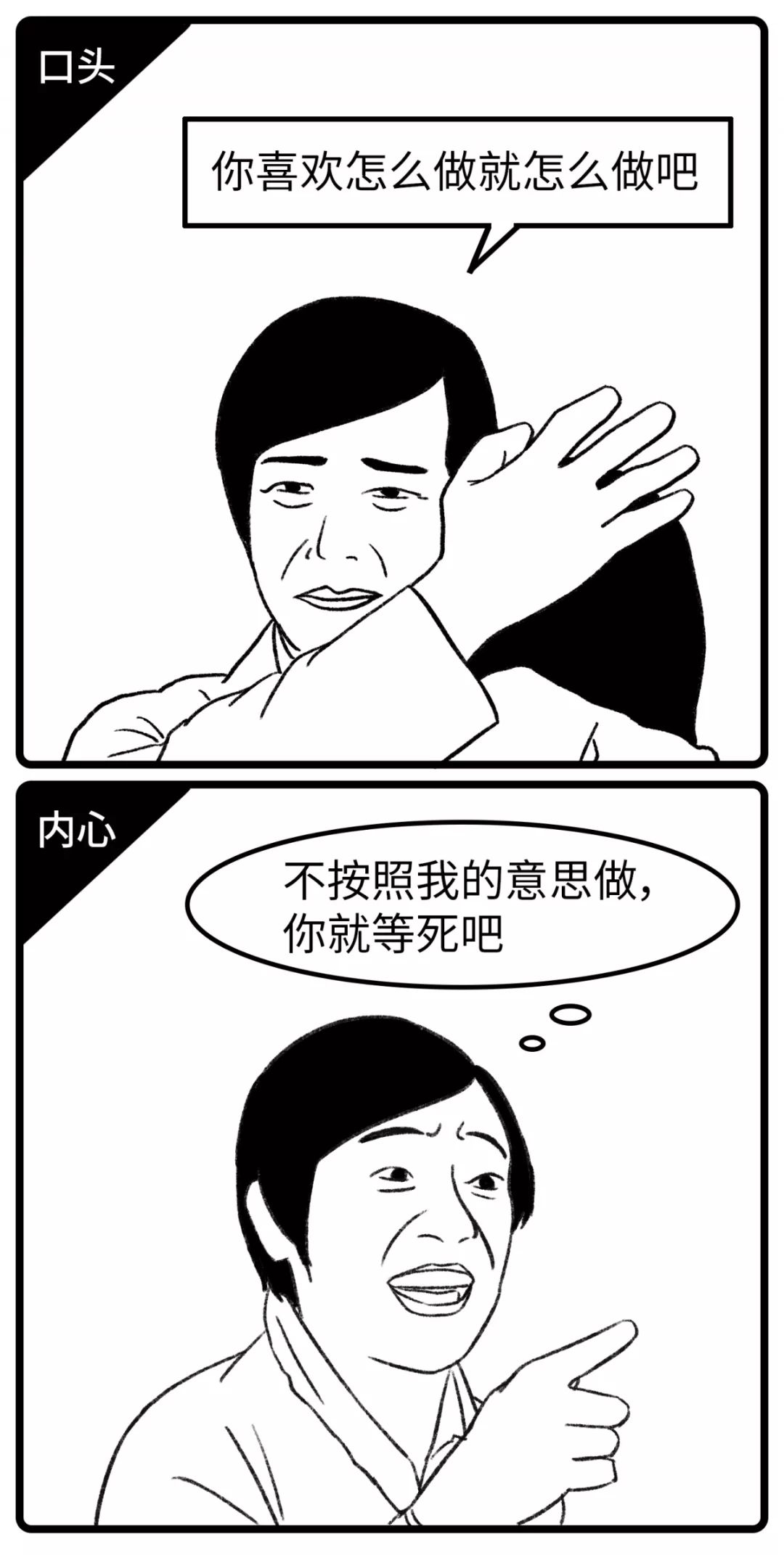45 这个事再说吧

=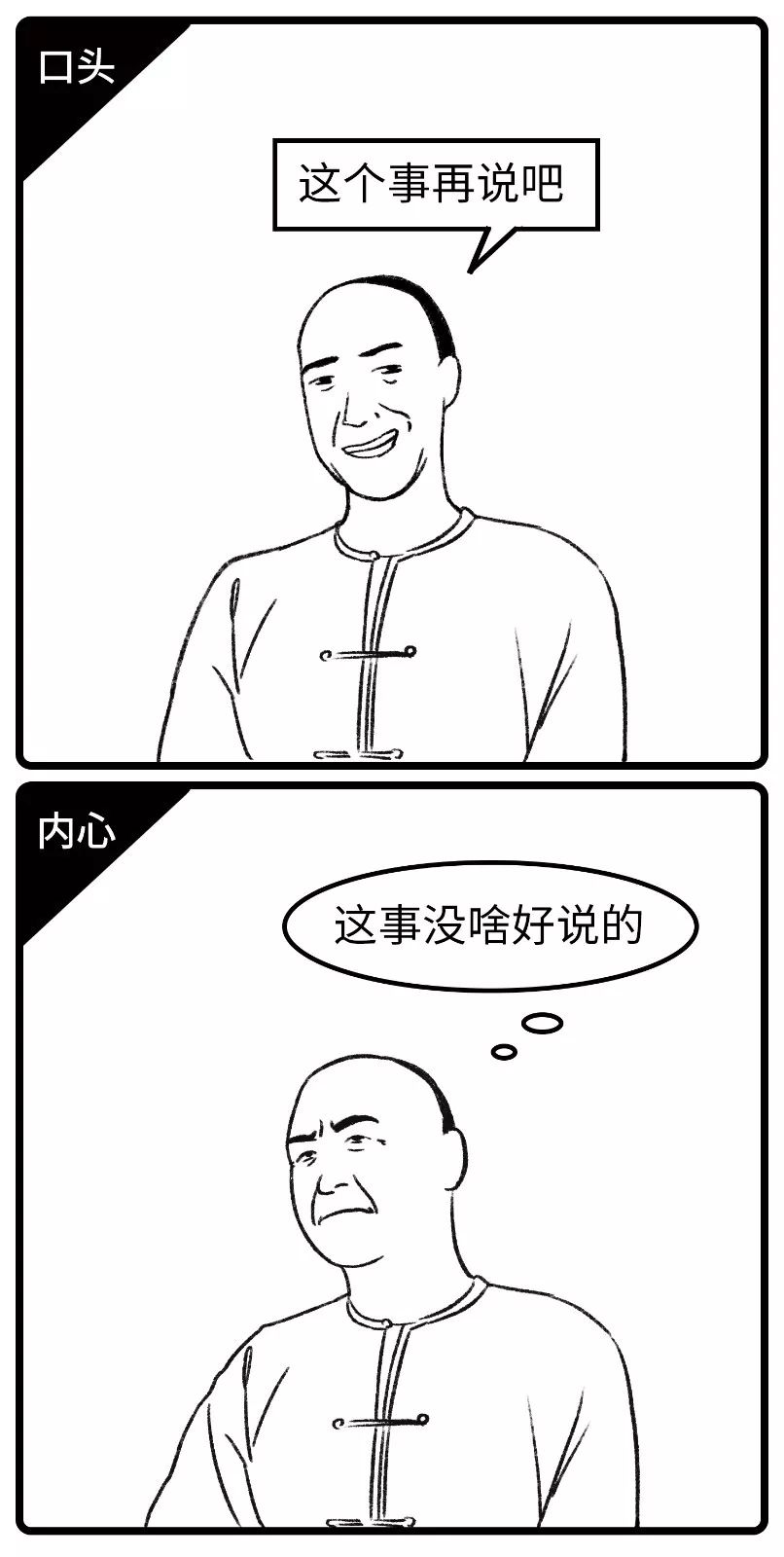46 我再想想

=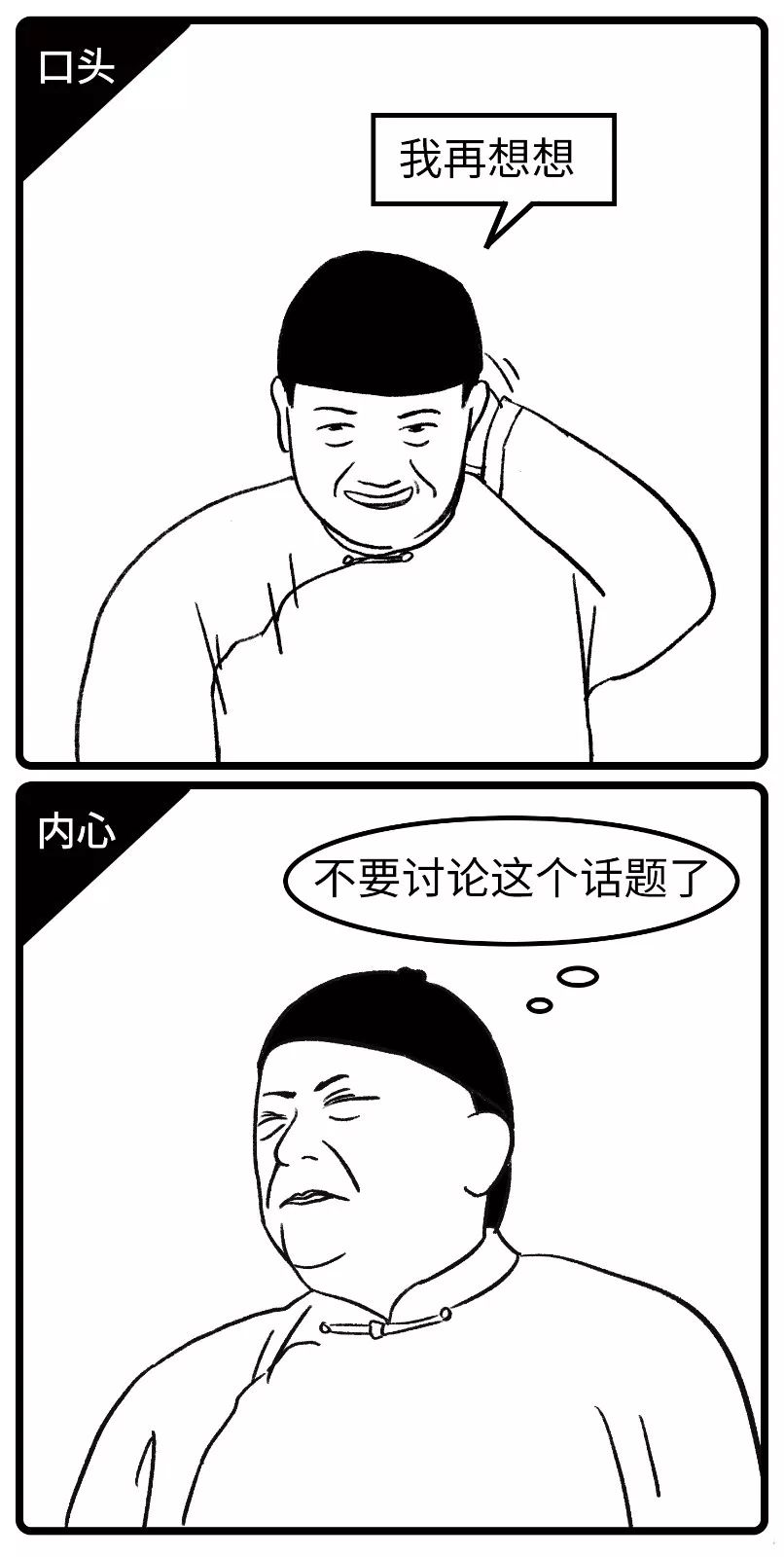47 你是个实在人

=48 这么晚了，干脆留下来吃饭吧?

=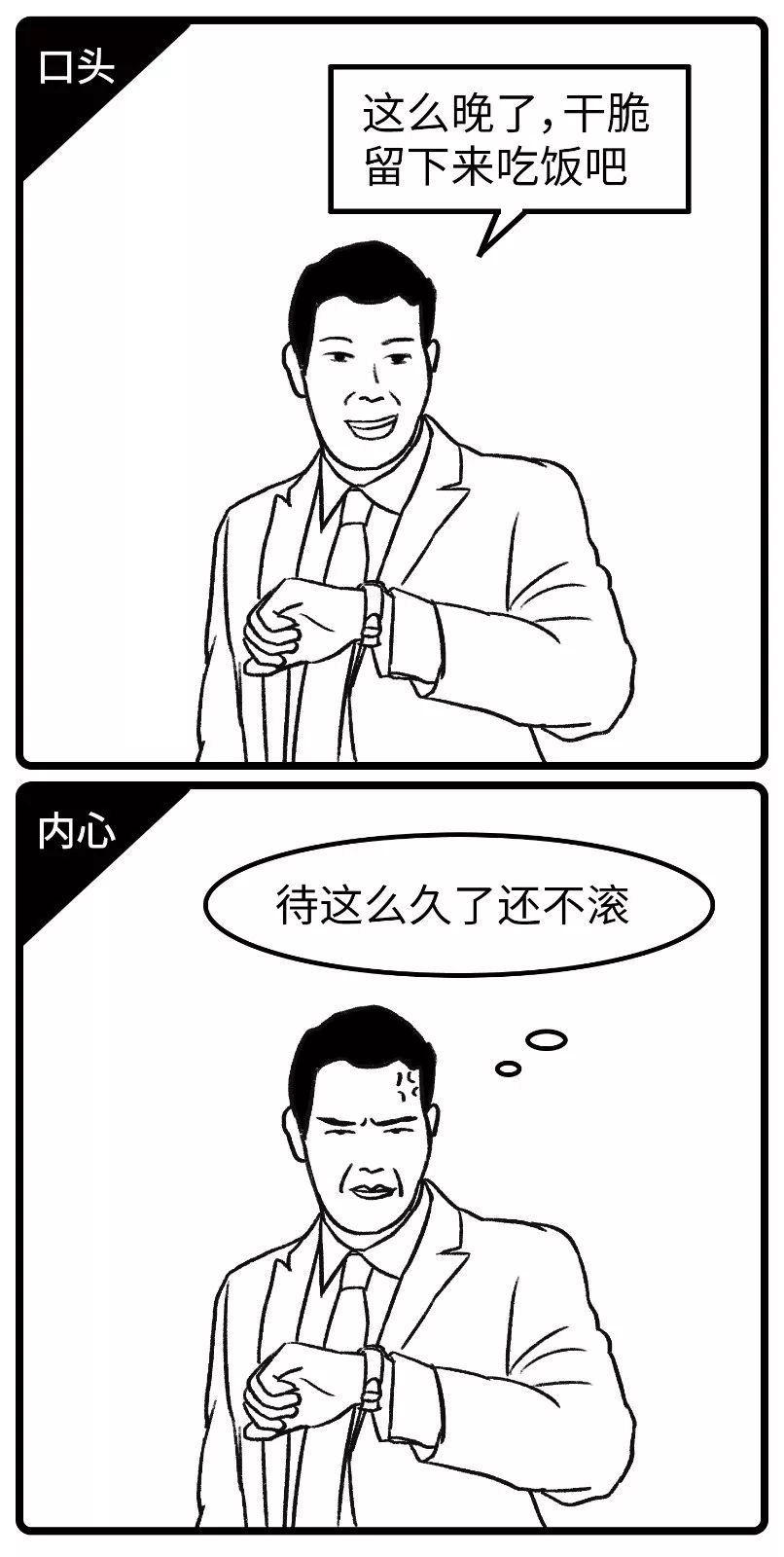49 有空一定去

=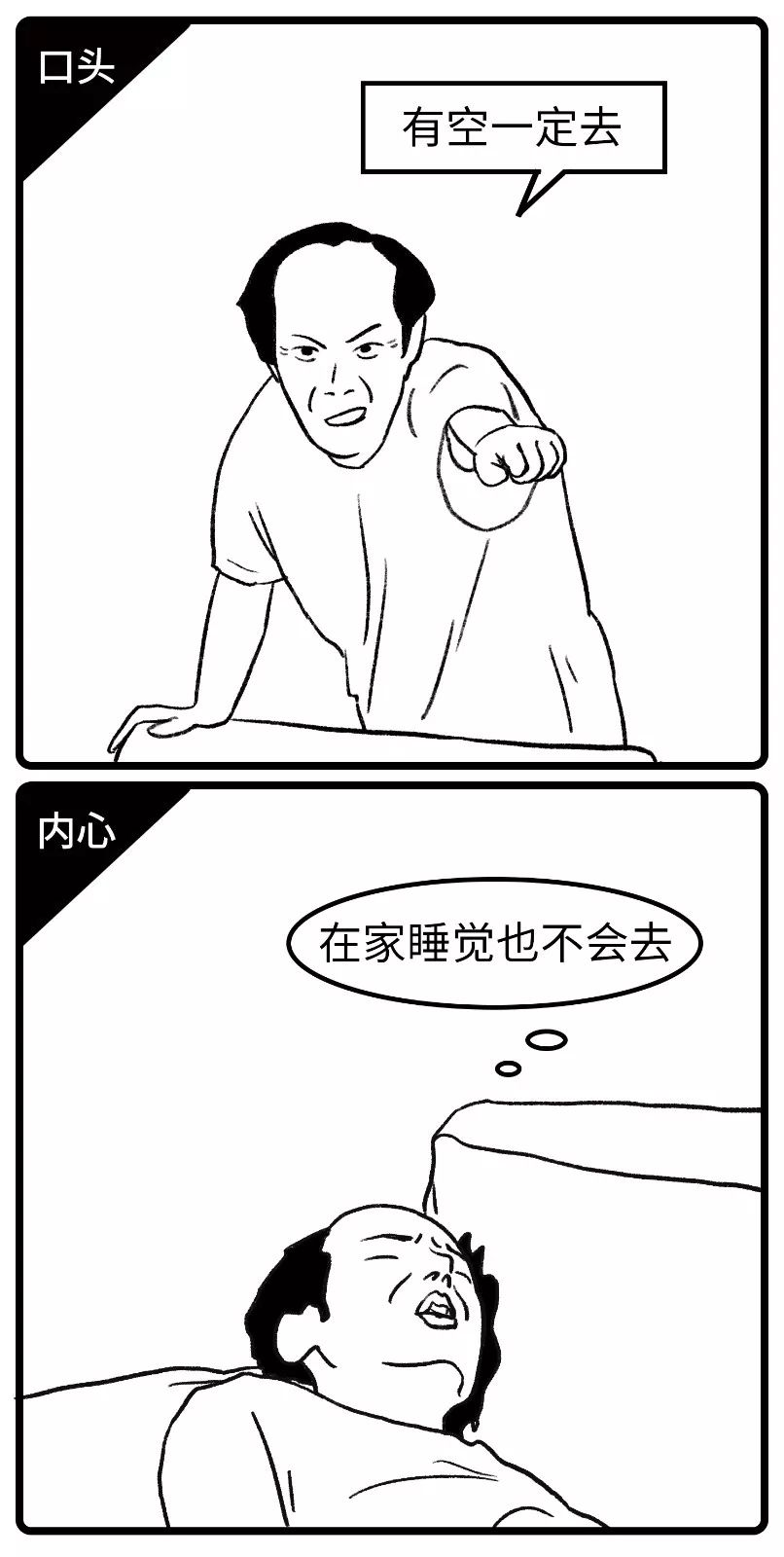50 哎呀，我不是这个意思

=